aaron67's log 花拳绣腿 & 柴米油盐 2023-01-25T09:43:22.864Z https://aaron67.cc/ aaron67 Hexo 收款方代付交易矿工费的解决方案 https://aaron67.cc/2022/04/04/solutions-for-the-payee-to-pay-the-transaction-fee/ 2022-04-04T00:00:00.000Z 2023-01-25T09:43:22.864Z 对于 Alice 发送 BSV 给 Bob 这种常见的使用场景，交易的矿工费一般是由 Alice 也就是交易的发送方支付的。而对于 BIP-270 所描述的支付场景，商户也就是收款方可能更愿意承担这部分费用，替用户支付交易的矿工费来尽可能的促成交易或提升用户体验。

Alice 去店里买咖啡，需要支付 1 BSV，她会将签好名的付款交易直接发给 Bob，然后等待；Bob 收到 Alice 发来的交易后确认交易有效，然后交付商品，流程结束。在整个交互过程中，Bob 会关心自己是否真的收到了钱，所以他会把 Alice 的交易迅速发给矿工来验钞和“结算”；而 Alice 只关心自己是否能尽快取走咖啡，以及自己的支付找零是否安全。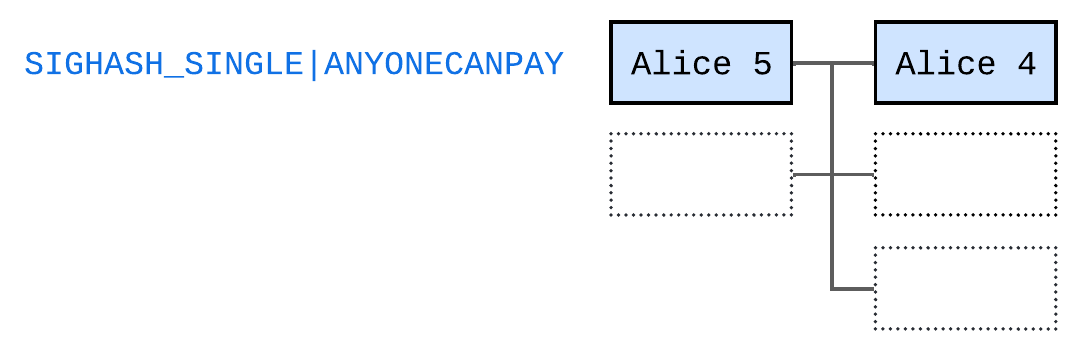Bob 收到这笔交易后，会先添加 1 BSV 咖啡钱的输出（绿色）给自己，然后添加 gas 的输入和找零（红色），并使用 SIGHASH_ALL 标记签名。Bob 最终发给矿工的交易如下图所示。• Alice 如果有多个输入要怎么签名
• Bob 如何提供 gas 输入来避免性能瓶颈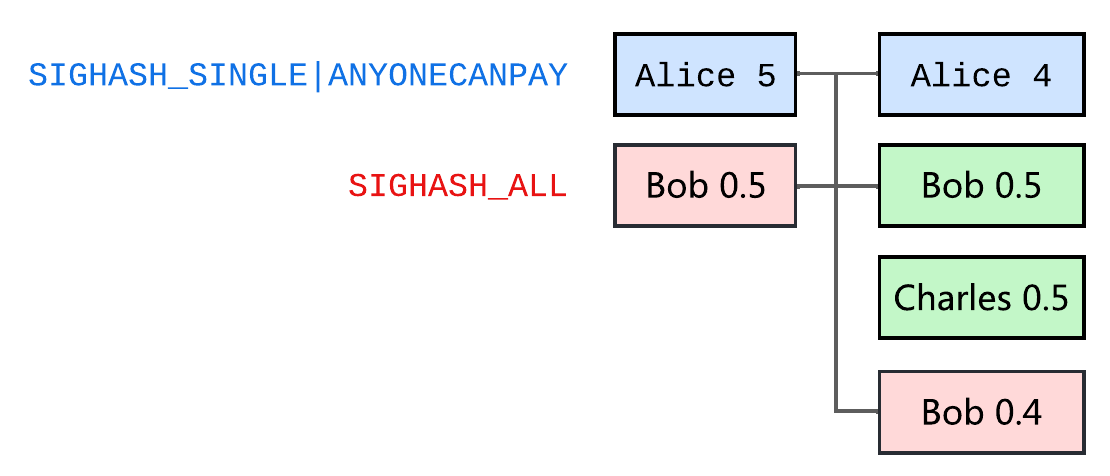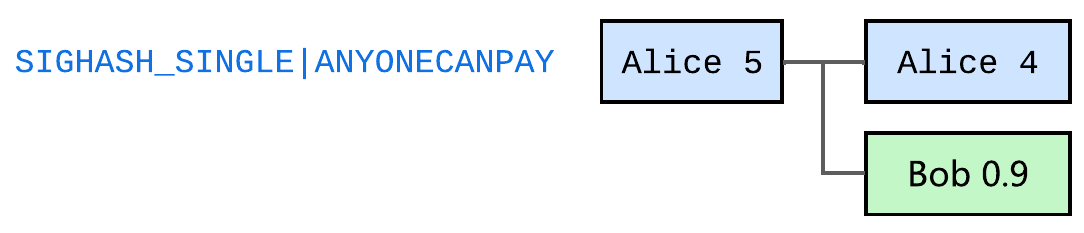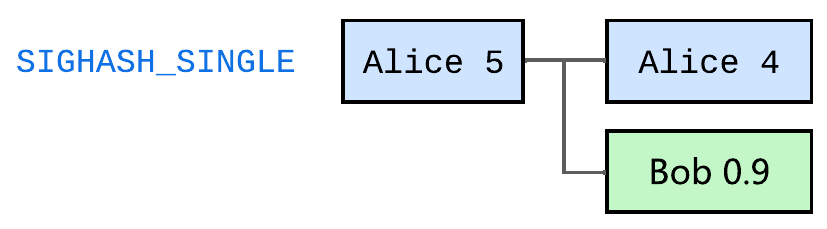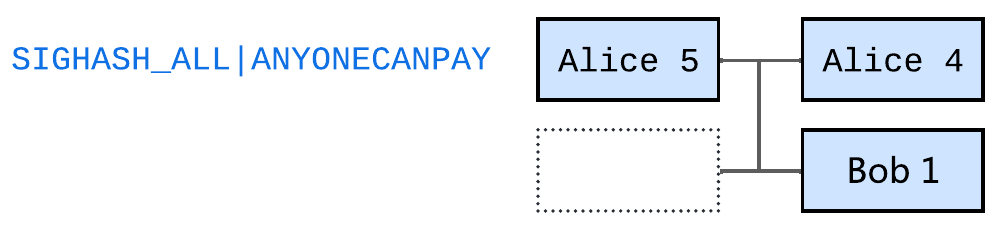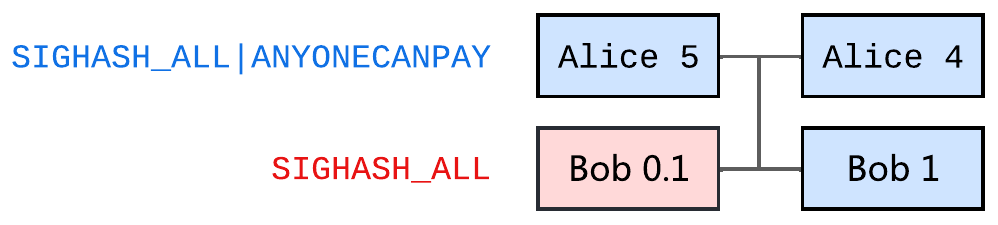Bob 还是可以用上文提到的方法提前拆分和管理 gas UTXO，但在每次取队列后，都立即花费拿到的 gas UTXO（红色）新生成一个特定金额的输出（黄色）来作为这次付款交易的 gas 输入。因为 Bob 只会对收到的付款交易添加 1 个输入，所以他同样能提前精确的算出最终交易需要多少矿工费。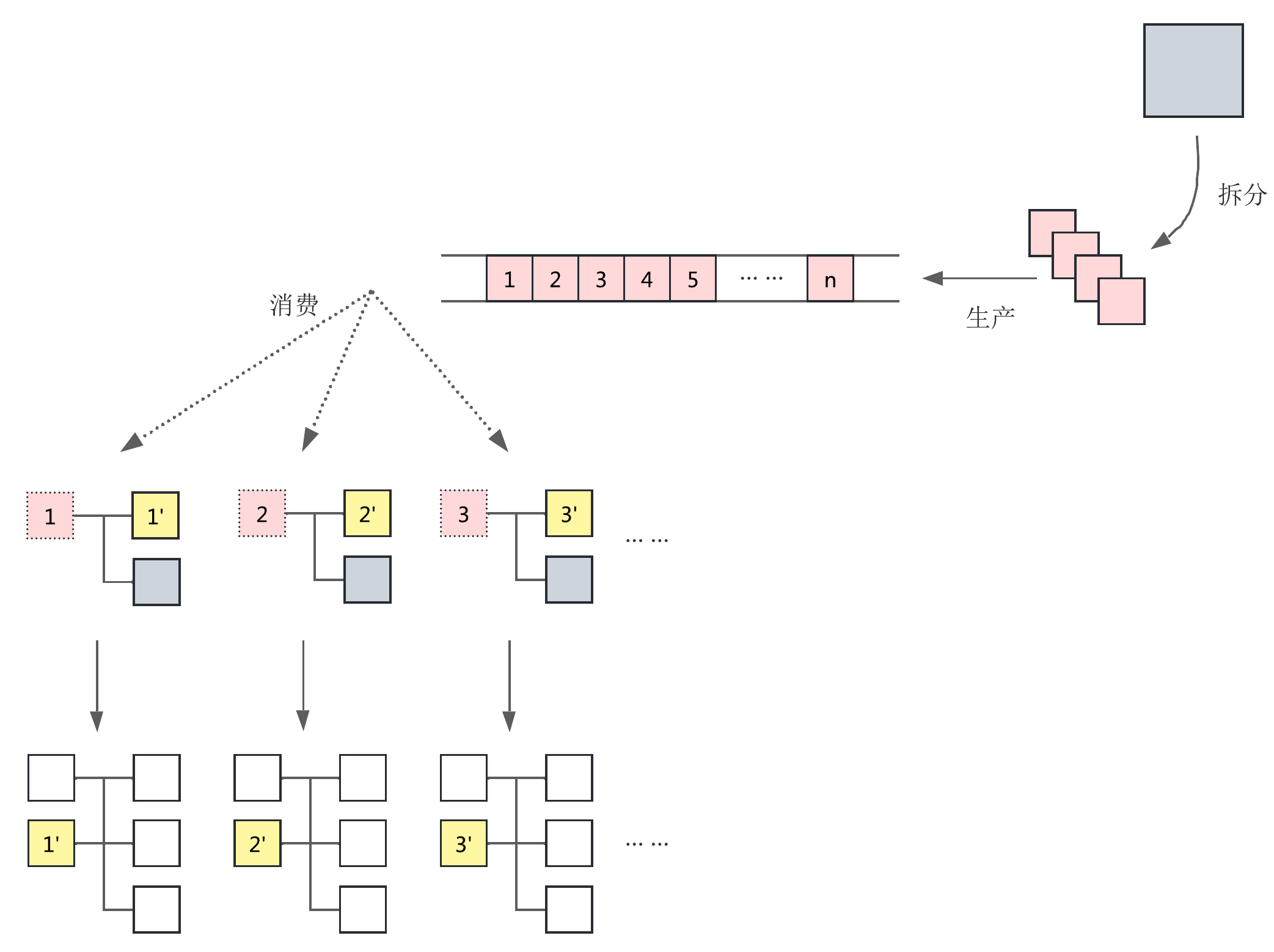# 参考

]]>
<p>对于 Alice 发送 BSV 给 Bob 这种常见的使用场景，交易的矿工费一般是由 Alice 也就是交易的发送方支付的。而对于 <a href="https://github.com/moneybutton/bips/blob/master/bip-0270.mediawiki" target="_blank" rel="noopener">BIP-270</a> 所描述的支付场景，商户也就是收款方可能更愿意承担这部分费用，替用户支付交易的矿工费来尽可能的促成交易或提升用户体验。</p> <p>本文将针对收款方如何代付 BSV 交易的矿工费，给出可行的解决方案。</p>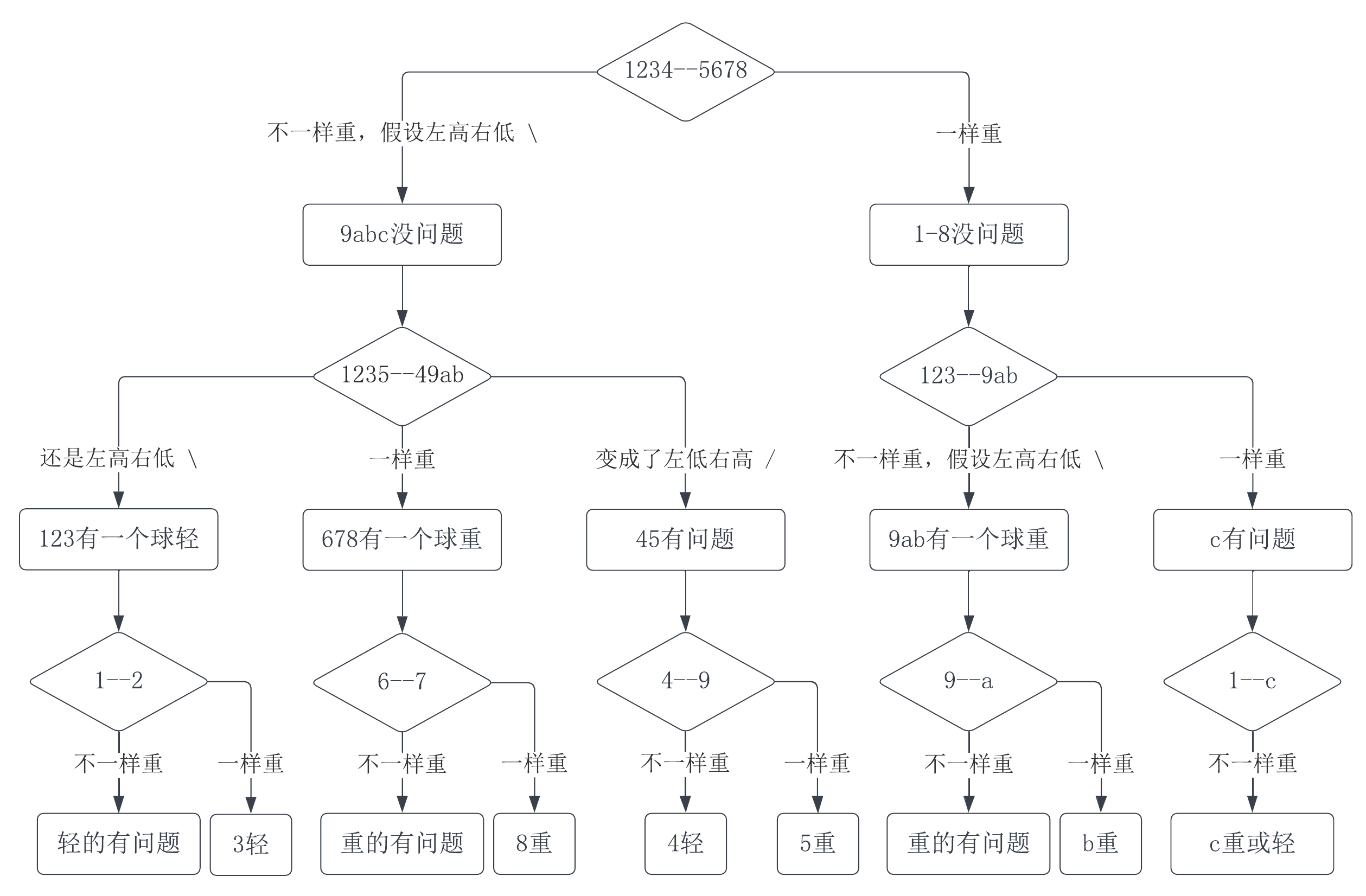# 结论一

$$\begin{cases}1 \le a + b \le 3 \\[0.5em]1 \le n - 2(a + b) \le 3\end{cases}$$

# 结论二

$$\frac{3^i + 1}{2} = \frac{3^i - 1}{2} + 1$$

• 称 ① 和额外的正常球：
• 不平衡，则确定 ① 是问题球并能确定它是偏轻还是偏重
• 平衡，则“排除”出 ② 是问题球，但无法确定它是偏轻还是偏重

• 称 ①、② 和 ③、正常球
• 不平衡，根据结论一再称一次就能从 ① ② ③ 中确定问题球并确定它是偏轻还是偏重
• 平衡，称 ④ 和正常球
• 不平衡，则确定 ④ 是问题球并能确定它是偏轻还是偏重
• 平衡，则“排除”出 ⑤ 是问题球，但无法确定它是偏轻还是偏重

# 结论

• 不平衡，根据结论一，再称 $k-1$ 次在最坏情况下最多可以有 $3^{k-1}$ 个都“上过天平”的球。但 $3^{k-1}$ 是个奇数，而每次拿到天平两边称的球加一起肯定是偶数个，所以这个分支实际最多可以有 $3^{k-1} - 1$ 个球
• 平衡，则能确定天平上的都是正常球，至少有 1 个，根据结论二，剩下的球最多可以有 $\cfrac{3^{k-1} - 1}{2}$ 个

# 通解方案

• 左轻右重，则 ⑨ ⑩ ⑪ ⑫ 是正常球，① ② ③ ④ “可能偏轻”，⑤ ⑥ ⑦ ⑧ “可能偏重”。把这 8 个球分成三堆各 3、3、2 个球，每堆都不超过 3 个，前两堆各有两个球“可能偏轻”一个球“可能偏重”，称 ① ② ⑤ 和 ③ ④ ⑥
• 左轻右重，则 ③ ④ ⑤ ⑦ ⑧ 是正常球，① ② “可能偏轻”，⑥ “可能偏重”，称 ① 和 ②
• 左轻右重，则 ① 是问题球，偏轻
• 左重右轻，则 ② 是问题球，偏轻
• 平衡，则 ⑥ 是问题球，偏重
• 左重右轻，则 ① ② ⑥ ⑦ ⑧ 是正常球，③ ④ “可能偏轻”，⑤ “可能偏重”，称 ③ 和 ④
• 左轻右重，则 ③ 是问题球，偏轻
• 左重右轻，则 ④ 是问题球，偏轻
• 平衡，则 ⑤ 是问题球，偏重
• 平衡，则 ① ~ ⑥ 是正常球，⑦ ⑧ “可能偏重”，称 ⑦ 和 ⑧
• 左轻右重，则 ⑧ 是问题球，偏重
• 左重右轻，则 ⑦ 是问题球，偏重
• 平衡，不可能出现这种结果
• 左重右轻，则 ⑨ ⑩ ⑪ ⑫ 是正常球，① ② ③ ④ “可能偏重”，⑤ ⑥ ⑦ ⑧ “可能偏轻”。跟左轻右重实际等价，称 ① ② ⑤ 和 ③ ④ ⑥
• 平衡，则 ① ~ ⑧ 是正常球，在 ⑨ ⑩ ⑪ ⑫ 里确定问题球并确定它是偏轻还是偏重，需要称 2 次。根据结论二，一边放 $\cfrac{3^1 + 1}{2} = 2$ 个球，另一边放 1 个球和额外的 1 个正常球，称 ⑨ ⑩ 和 ⑪ ①
• 左轻右重，则 ⑫ 是正常球，⑨ ⑩ “可能偏轻”，⑪ “可能偏重”，称 ⑨ 和 ⑩
• 左轻右重，则 ⑨ 是问题球，偏轻
• 左重右轻，则 ⑩ 是问题球，偏轻
• 平衡，则 ⑪ 是问题球，偏重
• 左重右轻，则 ⑫ 是正常球，⑨ ⑩ “可能偏重”，⑪ “可能偏轻”。跟左轻右重实际等价，称 ⑨ 和 ⑩
• 平衡，则 ⑨ ⑩ ⑪ 是正常球，⑫ 被“排除”成问题球但不确定它是偏轻还是偏重，称 ⑫ 和 ①
• 左轻右重，则 ⑫ 是问题球，偏轻
• 左重右轻，则 ⑫ 是问题球，偏重
• 平衡，不可能出现这种结果

# 参考

]]>
<p>抖音上看到的面试题：</p> <p>有 12 个外观一样的小球，只知道其中一个球的重量与其它球不同。用一个没有砝码的天平，最坏情况下最少要称几次，才能找到这个重量不一样的小球并确定它是偏轻还是偏重。</p> <p>正确答案 3 次。</p> <div style="width: 75%; margin: auto"><img src="https://aaron67-public.oss-cn-beijing.aliyuncs.com/202203220419977.png" alt></div>

• 任何人都可以从合约中提取（withdraw）固定数量的 BSV，但连续两次提取必须至少间隔一定的冷却（cool down）时间
• 任何人都可以随时向合约中充值（deposit）
• 部署合约的人可以随时销毁（destroy）合约

# withdraw

1. 在合约中记录最后一次提取资金的时间 lastWithdrawTimestamp，这样就可以限制下一次提取必须在时间 lastWithdrawTimestamp + withdrawIntervals 之后
2. 要求调用交易 nLocktime 字段的值，不小于 lastWithdrawTimestamp + withdrawIntervals。如果满足，就把合约中记录的最后一次提款时间，更新为该交易 nLocktime 的值
3. 限制调用交易只能有两个输出，合约本身的输出和用户提款的 P2PKH

# 参考

]]>
<p>本文将实现一个运行在 BSV 链上的“水龙头”（faucet）合约，它可以满足以下需求：</p> <ul> <li>任何人都可以从合约中提取（withdraw）固定数量的 BSV，但连续两次提取必须至少间隔一定的冷却（cool down）时间</li> <li>任何人都可以随时向合约中充值（deposit）</li> <li>部署合约的人可以随时销毁（destroy）合约</li> </ul>
BSV 的 nLocktime 和 nSequence https://aaron67.cc/2022/03/12/bsv-nlocktime-nsequence/ 2022-03-12T00:00:00.000Z 2023-01-25T09:43:22.861Z BSV 的创世纪升级，恢复了交易的 nLockTime 字段和交易输入的 nSequence 字段的原始功能。这两个字段会共同决定交易的“最终性”（finality）。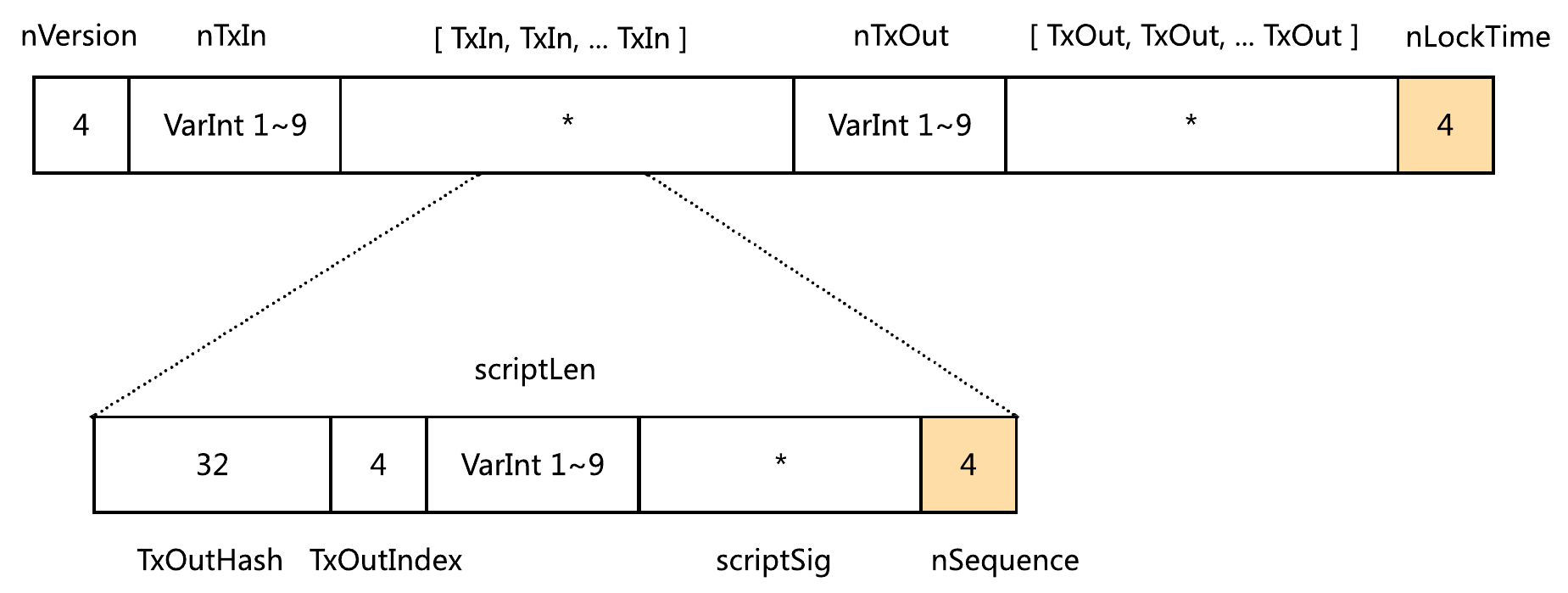• 如果输入 nSequence 的值等于 0xFFFFFFFF，那么这个输入是 final 的
• 如果输入 nSequence 的值小于 0xFFFFFFFF，那么这个输入是 non-final 的
• 如果交易的所有输入都是 final 的，那么这个交易就是 final 的。此时不论 nLocktime 的值是多少，都会被忽略
• 如果交易至少有一个 non-final 输入：
• 如果 nLocktime 小于 500,000,000，那么它的值表示一个区块高度
• 如果当前区块高度不小于这个值，那么这个交易是 final 的
• 否则，这个交易是 non-final 的
• 如果 nLocktime 不小于 500,000,000，那么它的值表示一个 Unix 时间戳
• 如果当前 MTP 时间不小于这个值，那么这个交易是 final 的
• 否则，这个交易是 non-final 的

1. 等待，直到满足 nLocktime 的限制
2. 被“新版本的自己”替换

• 新交易与之前收到的 non-final 交易具有相同顺序的相同输入
• 新交易中每个输入 nSequence 的值都不小于 non-final 交易中对应输入 nSequence 的值
• 新交易中至少有一个输入 nSequence 的值大于 non-final 交易中对应输入 nSequence 的值

# 参考

]]>
<p>BSV 的<a href="https://github.com/bitcoin-sv-specs/protocol/blob/master/updates/genesis-spec.md" target="_blank" rel="noopener">创世纪升级</a>，恢复了<strong>交易的</strong> nLockTime 字段和<strong>交易输入的</strong> nSequence 字段的原始功能。这两个字段会<strong>共同决定</strong>交易的“最终性”（finality）。</p> <p><img src="https://aaron67-public.oss-cn-beijing.aliyuncs.com/202203122348671.png" style="zoom:35%;"></p>

1. 有一个 UTXO 被称作计数器合约，状态初始值为 0
2. 该 UTXO 被花费时，必须生成一个新的计数器 UTXO，并将之前的状态值加 1 记录到新的 UTXO 中
3. 规则  由矿工验证，如果没有生成符合要求的新 UTXO，就无法花费当前这个 UTXO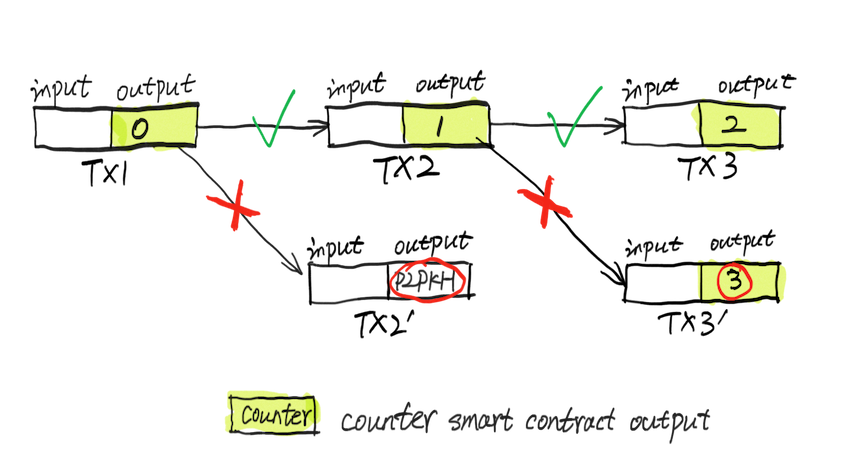# v1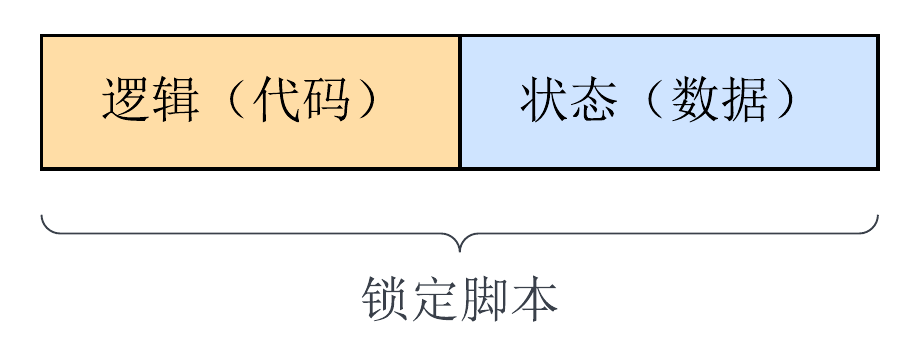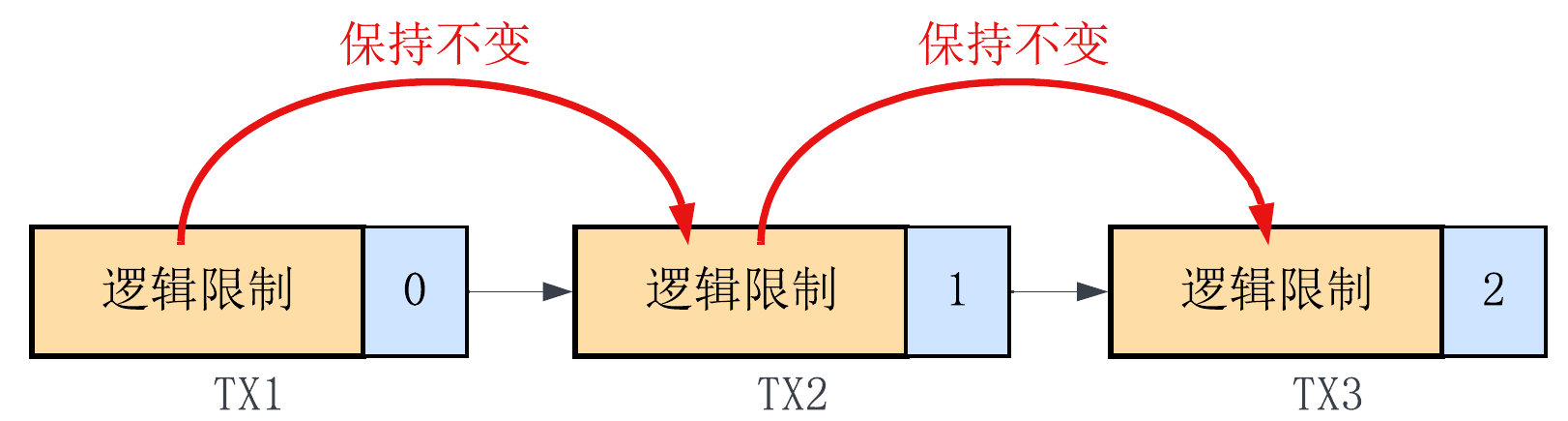1. 调用 Tx.checkPreimage 函数来保证传入的 txPreimage 确实是当前交易的 sighash preimage
2. 从 txPreimage 中解析出当前 UTXO 锁定脚本的原文，从而得到代码段（黄色）和数据段（蓝色）的内容
3. 根据当前状态计算正确的新状态
4. 验证当前交易的输出：构造出我们期望的当前交易的所有输出，要求它们的 SHA256 双哈希（hash256(expectedOutput)）跟 txPreimage 中的 hashOutputs（SigHash.hashOutputs(txPreimage)）保持一致

# v2

1. 验证 txPreimage
2. 处理数据
3. 验证当前交易的输出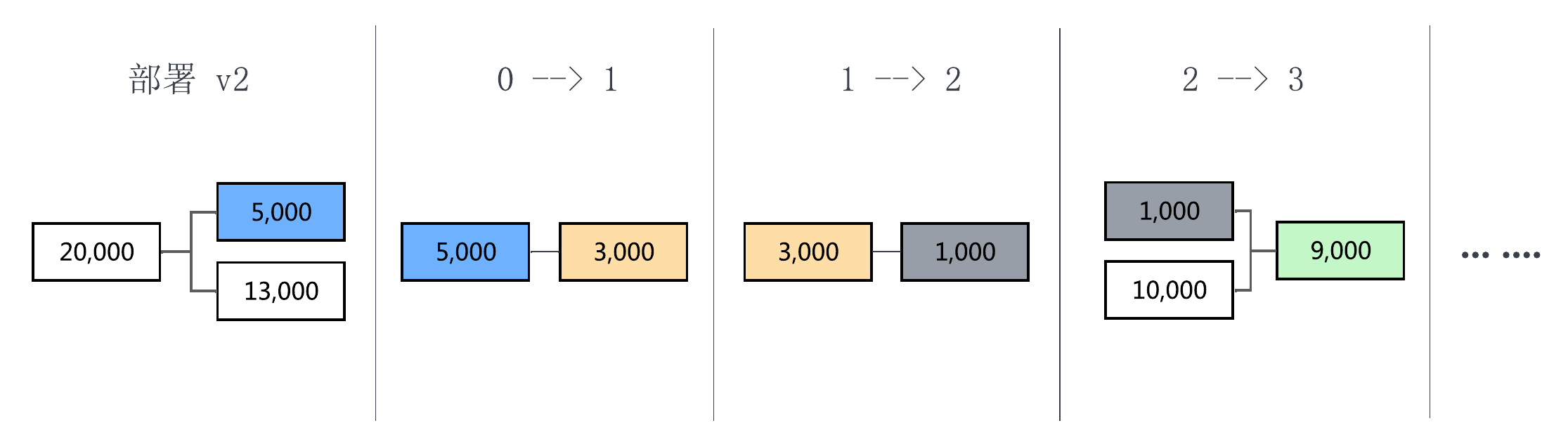# v3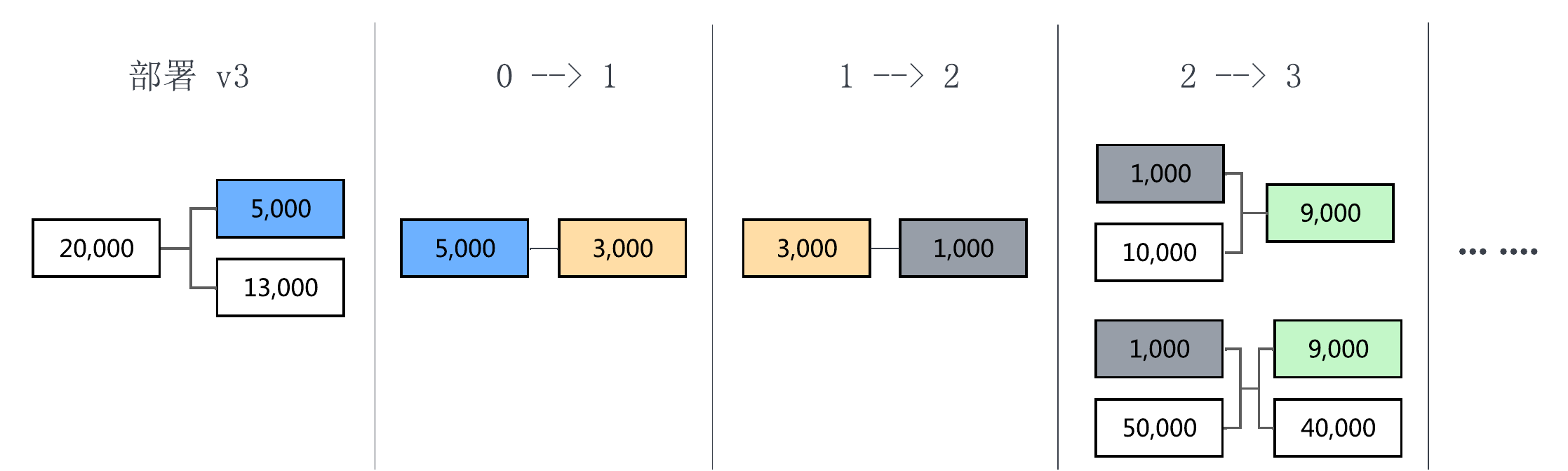# v4

1. 调用 Tx.checkPreimageSigHashType 函数并传入指定的 sigHashType 来验证 preimage 的真实性
2. 新的合约输出与之前锁定同样数量的 BSV

# 总结

• 要想实现“有状态”的合约就必须使用 preimage（作为参数传入解锁方法），因为要获取当前 UTXO 的锁定脚本，并限制新产生 UTXO 的锁定脚本
• sighash preimage 中与交易输出有关的项只有 hashOutputs，它是所有交易输出（SIGHASH_ALL）或与当前输入相对应的交易输出（SIGHASH_SINGLE）的 SHA256 双哈希，也可能是 32 字节的 0x00（SIGHASH_NONE）
• 因为 hashOutputs 是一个哈希值，所以我们必须要能在解锁方法中完全确定当前交易（需要被限制）的所有输出，才可以计算出期望的 hashOutputs，从而通过限制它跟 txPreimage 中 hashOutputs 的值保持一致来控制交易输出
]]>
<p>在文章<a href="/2022/03/06/bsv-stateful-utxo-and-op-push-tx/" title="有状态的 UTXO 和 OP_PUSH_TX 的技术原理">有状态的 UTXO 和 OP_PUSH_TX 的技术原理</a>中，我们提到了计数器合约，它可以把自己被调用的次数记录在链上，具体为：</p> <ol> <li>有一个 UTXO 被称作计数器合约，状态初始值为 0</li> <li>该 UTXO 被花费时，必须生成一个新的计数器 UTXO，并将之前的状态值加 1 记录到新的 UTXO 中</li> <li>规则  由矿工验证，如果没有生成符合要求的新 UTXO，就无法花费当前这个 UTXO</li> </ol> <p>本文将介绍该合约 5 个不同版本的实现，带你了解如何使用 <a href="https://scryptdoc.readthedocs.io/en/latest/" target="_blank" rel="noopener">sCrypt</a> 在 BSV 上开发智能合约。</p> <p><img src="https://aaron67-public.oss-cn-beijing.aliyuncs.com/202203062348930.png" alt></p>
BSV 交易签名相关的技术名词 https://aaron67.cc/2022/03/09/technical-terms-related-to-bsv-transaction-signature/ 2022-03-09T00:00:00.000Z 2023-01-25T09:43:22.864Z 在介绍 BSV 交易的签名时，出现了不少技术名词。可能有一些历史原因、翻译的差别，抑或是习惯叫法的不同，导致大家给同样的东西起了不同的名字。这虽没有大的问题，但十分影响交流。

# 像和原像

$$hash(m) = d$$

• $d$ 是 $m$ 的（image）
• $m$ 是 $d$ 的原像（preimage）

# 交易摘要和交易原像

• SIGHASH type(s)
• Signature type(s)
• Signature Hash type(s)

ECDSA 算法会对消息做哈希。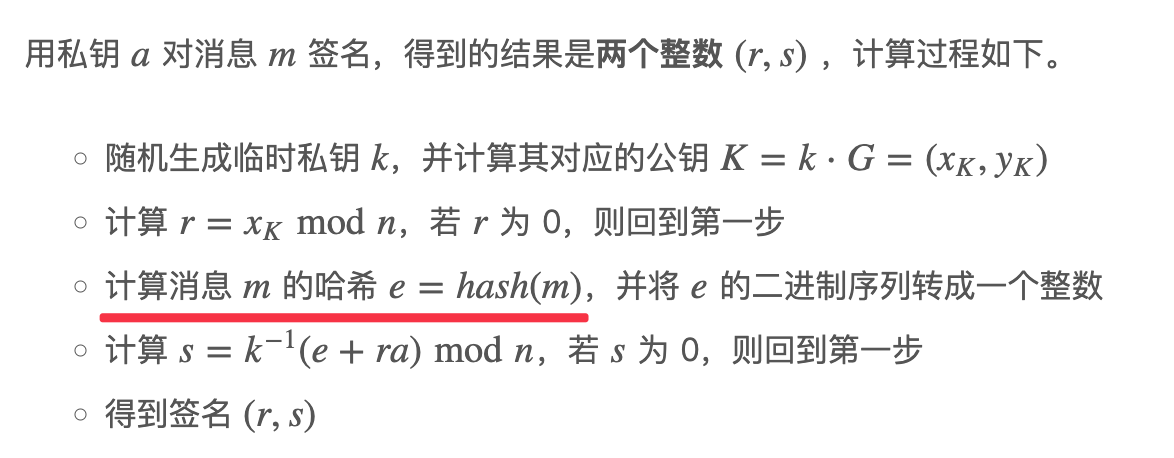$$hash256(交易摘要) = sighash$$

sighash 是 SignatureHash 的缩写。在比特币节点的早期实现中有一个叫 SignatureHash 的函数，用于根据交易输入和当前交易计算要签名的消息对应的哈希，所以常用 sighash 来表示这个函数的计算结果。

BSV 交易原像的一些历史：

• 在很久以前，为了能对整个交易签名，会直接把序列化后的交易数据作为 sighash preimage 的一部分
• 后来提出了 BIP-143，改用 hashPrevouts、hashSequence 和 hashOutputs 三个摘要来简化
• 2017 年 Bitcoin Core（BTC）分叉时，Bitcoin Cash（BCH）在代码里再次修改了 SignatureHash 方法（新增值为 0x40 的 FORKID）来避免重放攻击（replay attack）。对之前没有 FORKID 的交易（之前交易的 FORKID 值相当于是 0），通过调用原来的方法来实现向后兼容（Backward Compatibility）
• BSV 和 BCH 目前使用的都是兼容 BIP-143 的 sighash preimage 算法

# 致谢

]]>
<p>在介绍 BSV 交易的签名时，出现了不少技术名词。可能有一些历史原因、翻译的差别，抑或是习惯叫法的不同，导致大家给同样的东西起了不同的名字。这虽没有大的问题，但十分影响交流。</p> <p>本文将试着对签名相关的技术名词做一个梳理和对应。</p>

# UTXO 模型

BSV 的交易会消耗一个或多个输入，同时产生一个或多个输出。交易（除了 Coinbase 交易）的输入，都是之前某笔交易的输出。如果某个交易输出当前是未花费（unspent）的状态，也就是这个交易输出当前还没有被某个交易拿去作为交易输入使用，我们就称之为未花费的交易输出（UTXO，Unspent Transaction Outputs）。这些（前序）交易未被花费的交易输出（UTXO），可以在之后，作为交易输入被花费。我们说，UTXO 是构成交易的基本元素，因为每笔交易（除了 Coinbase 交易）都是在不断地消耗已存在的 UTXO，同时产生新的 UTXO。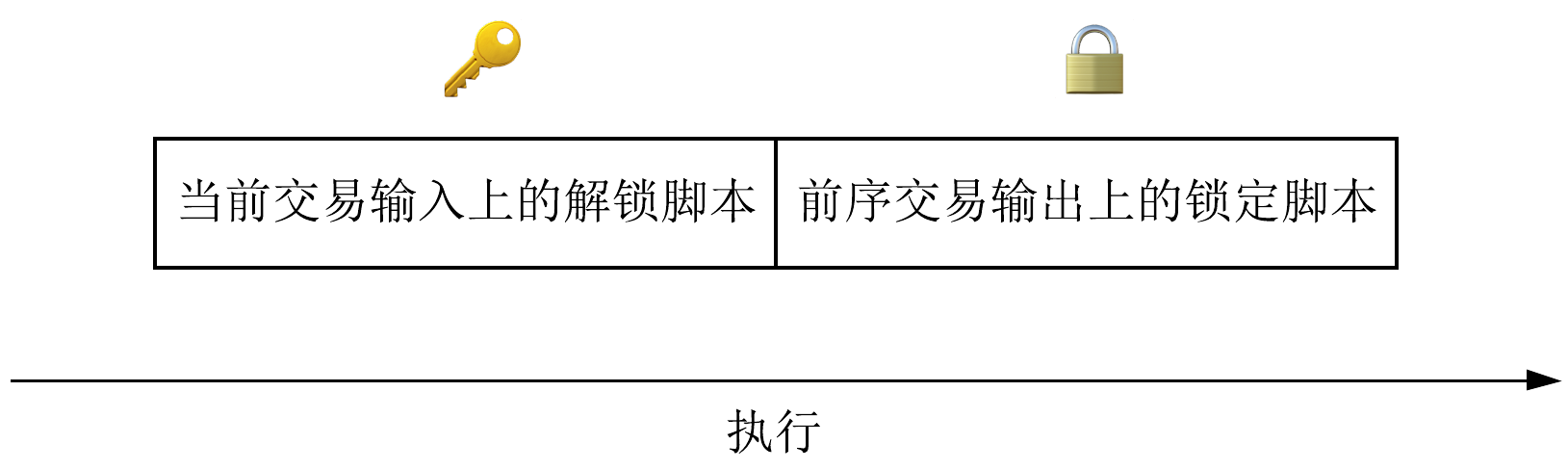BVM 验证脚本就像是在调用 unlock 函数，如果返回结果为真则验证通过，否则失败。# “有状态”的 UTXO

1. UTXO 的锁定脚本里记录了状态
2. UTXO 的状态可以随着交易链条的流转按要求变化，且状态链条不会中断1. 有一个 UTXO 被称作“计数器”，状态初始值为 0
2. 该 UTXO 被花费时，必须生成一个新的“计数器” UTXO，并将之前的状态值加 1 记录到新的 UTXO 中
3. 规则  由矿工验证，如果没有生成符合要求的新 UTXO，就无法花费当前这个 UTXO# 交易的上下文

1. 这个 UTXO 在前序交易中的锁定脚本。用于获取逻辑限制和当前状态
2. 当前交易交易输出的锁定脚本。用于验证花费这个 UTXO 的交易所产生的新 UTXO 的锁定脚本，其逻辑是否与当前 UTXO 保持一致，其状态变化是否符合要求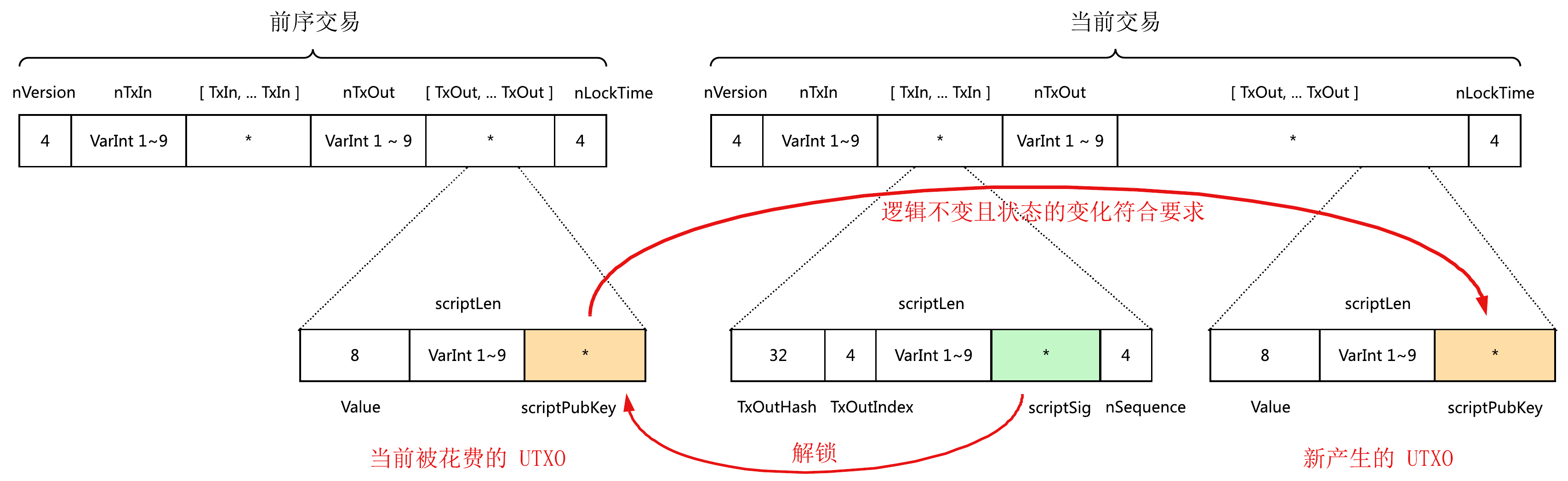• 第  项是该 UTXO 锁定脚本的原文，我们可以从中得到逻辑 L 和状态 S
• 第  项 hashOutputs 是当前交易交易输出的双哈希。这没有什么问题，我们只需要对逻辑 L 和正确的新状态 S’ 拼接后的内容做双哈希，保证结果与交易摘要中的 hashOutputs 一致即可。# OP_PUSH_TX 的技术原理

1. 从栈上弹出私钥和消息，执行 ECDSA 签名
2. 将计算出的签名数据重新压回栈中

# sCrypt

sCrypt 已经把这些技术都封装好了。

# 总结

• UTXO 被锁定脚本锁定，除非提供正确的解锁脚本，否则无法花费
• 对“有状态”的 UTXO，除了要在锁定脚本中记录状态外，还需要保证状态能在交易链条中保持。只有强制传递锁定脚本的逻辑才能保证状态链条不会中断
• 为了实现 UTXO 的状态延续，需要在解锁该 UTXO 时能获取到当前交易的上下文
• 把当前交易的交易摘要放到解锁脚本中解决了交易上下文的问题，但还需要利用“链上签名”技术和 OP_CHECKSIG 来保证传入交易摘要的真实性
• sCrypt 是 BSV 脚本的高级编程语言，可以用来编写复杂的脚本，它还封装了 OP_PUSH_TX 技术可以管理“有状态”的 UTXO，是你开发 BSV 智能合约的必备工具

# 参考

]]>
<p>一般都认为，UTXO 是“无状态”的。对于生活中常见的支付场景，币转出后就意味着失去了对这些币的“控制权”，收款人可以随意支配收到的 BSV。</p> <p>那 UTXO 可以做到“有状态”吗？</p> <p>本文将介绍“有状态” UTXO 的实现原理。请在继续阅读前，先理解 <a href="/2022/02/28/bsv-opcode-checksig-and-sighash-flags/" title="OP_CHECKSIG 操作码的工作方式">OP_CHECKSIG 操作码的工作方式</a>。</p>

• DER 格式序列化后的 ECDSA 签名
• SIGHASH 标记

# 交易摘要1. nVersion of the transaction，该交易的版本号，即 nVersion（4 字节小端）
2. hashPrevouts （32 字节）
3. hashSequence（32 字节）
4. outpoint，该输入引用的交易输出，即 TxOutHash（32 字节小端） 和 TxOutIndex（4 字节小端）的拼接
5. scriptCode of the input，该输入的锁定脚本，也就是这个输入在之前作为交易输出时的 scriptLen 和 scriptPubKey 的拼接
6. value of the output spent by this input，该输入的金额，也就是这个输入在之前作为交易输出时的 Value（8 字节小端）
7. nSequence of the input，该输入的 nSequence（4 字节小端）
8. hashOutputs（32 字节）
9. nLocktime of the transaction，该交易的 nLocktime（4 字节小端）
10. sighash type of the signature，该输入的 SIGHASH 标记（4 字节小端）

• 、 两项对应当前输入在前序交易中作为输出时的状态
• 、 两项对应当前输入在当前交易中作为输入时的状态。请注意这里是不包含解锁脚本的，因为签名最终会放到解锁脚本中，要签名的消息不能包含签名数据本身
•  对应当前输入的 SIGHASH 标记
• 、 两项对应当前交易的状态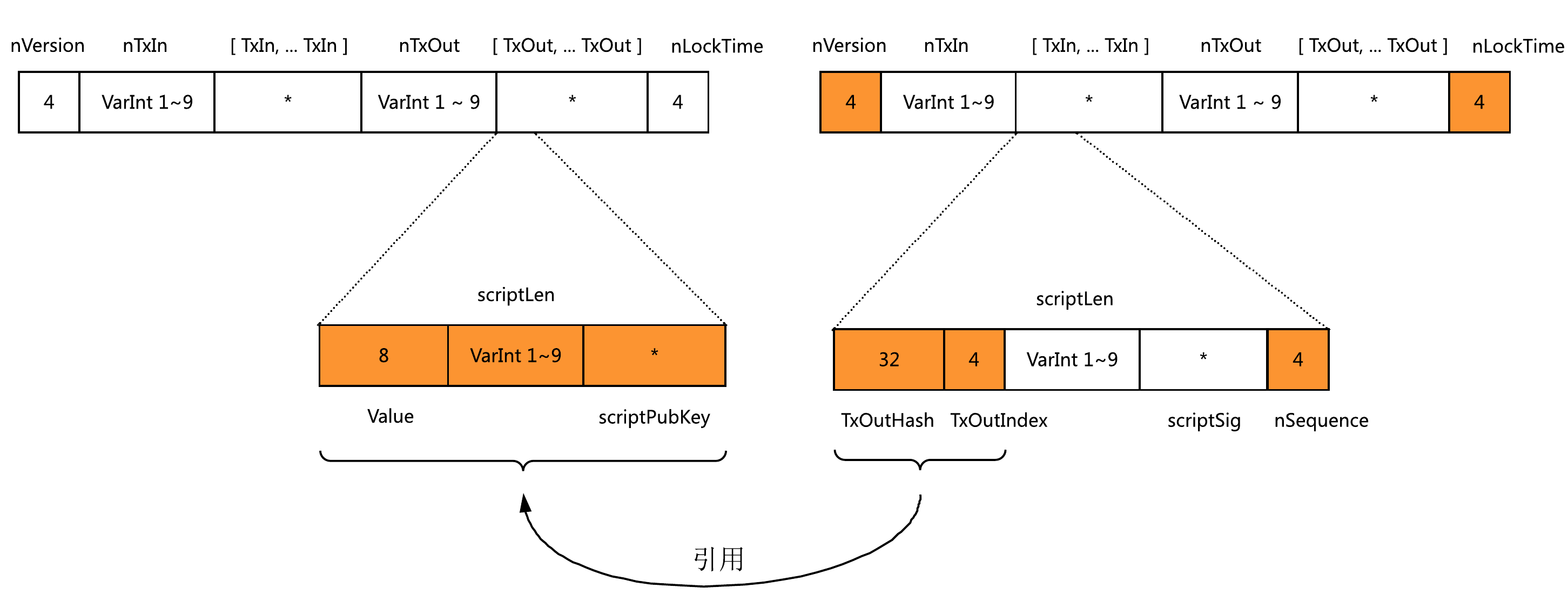# SIGHASH 标记

SIGHASH 标记一共有 6 种，由 4 个不同的项组合而成。

SIGHASH_ALL0x01
SIGHASH_NONE0x02
SIGHASH_SINGLE0x03
ANYONECANPAY0x80

SIGHASH 标记解释
SIGHASH_ALL0x01对所有输入、所有输出签名
SIGHASH_NONE0x02对所有输入、不对任何输出签名
SIGHASH_SINGLE0x03对所有输入、跟当前输入有相同序号的输出签名
SIGHASH_ALL | ANYONECANPAY0x81对当前输入、所有输出签名
SIGHASH_NONE | ANYONECANPAY0x82对当前输入、不对任何输出签名
SIGHASH_SINGLE | ANYONECANPAY0x83对当前输入、跟当前输入有相同序号的输出签名

• SIGHASH_ALL、SIGHASH_NONE 和 SIGHASH_SINGLE 会对所有的交易输入签名，它们的不同是对交易输出的限制
• ANYONECANPAY 则是对交易输入限制的放宽。如果包含这个标记，那么只对当前输入签名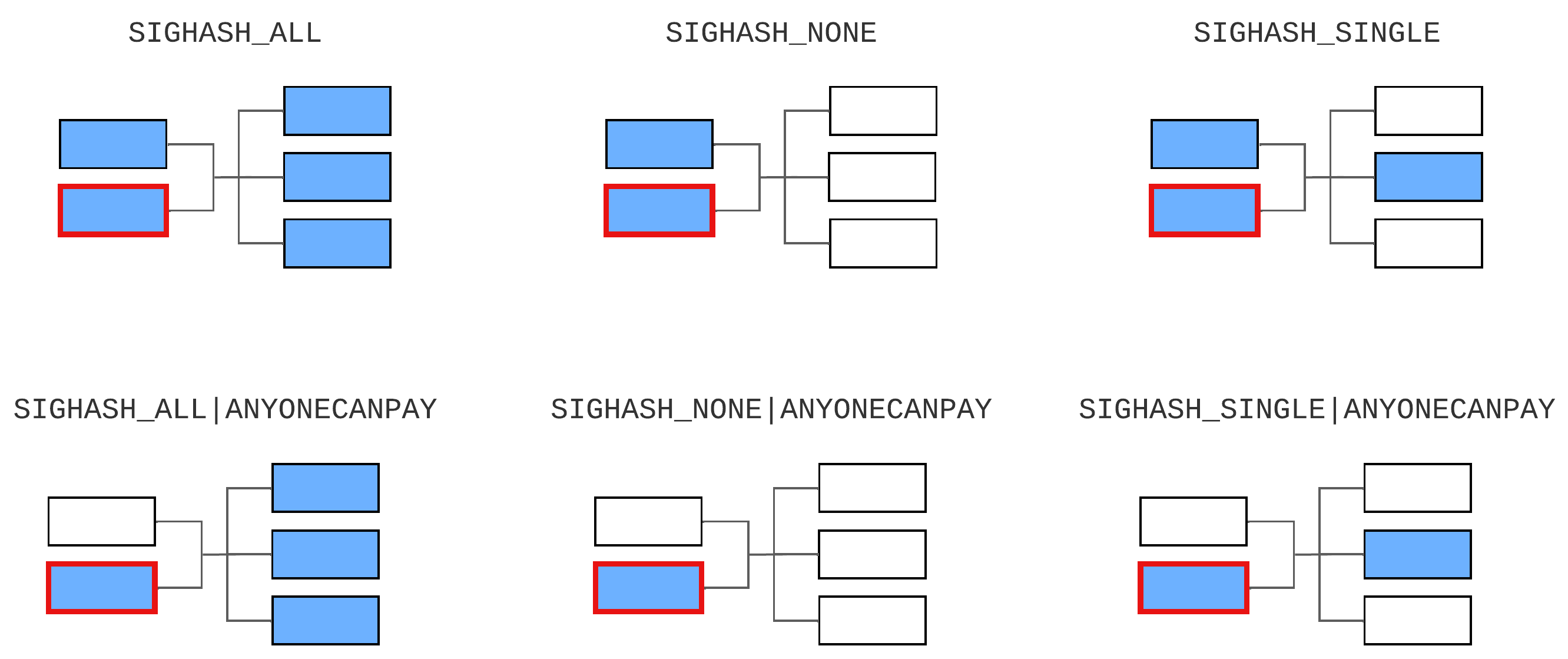• 交易摘要是针对交易输入来说的，不同的交易输入会对应不同的交易摘要，即使这些输入都使用相同的 SIGHASH 标记，它们的交易摘要也是不同的
• SIGHASH 标记作用在交易输入上，某个输入应该使用什么标记是由用户需求决定的，所有标记都没有使用限制可以随意选择。例如，你可以使用 SIGHASH_ALL | ANYONECANPAY 募集资金，使用 SIGHASH_NONE 签署空白的支票（任何人都可以添加交易输出）等。但请注意，对于含有多个输入的交易，所有 SIGHASH 标记最终反映到交易上的效果是“叠加”的。交易的一个输入使用 SIGHASH_ALL 另一个输入使用 SIGHASH_NONE 是没有意义的，它跟两个输入都使用 SIGHASH_ALL 效果一样

## hashPrevouts

• 如果 SIGHASH 标记不包含 ANYONECANPAY，则按顺序拼接所有输入的 outpoint（即交易摘要的 ）后做 SHA256 双哈希（double-SHA256）
• 否则，结果为 32 字节的 0x00

## hashSequence

• 如果 SIGHASH 标记不包含 ANYONECANPAY、SINGLE 和 NONE 三项中的任意一项（只能是 SIGHASH_ALL），则按顺序拼接所有输入的 nSequence 后做 SHA256 双哈希
• 否则，结果为 32 字节的 0x00

## hashOutputs

• 如果 SIGHASH 标记既不包含 SINGLE 也不包含 NONE（只能是 SIGHASH_ALL 或 SIGHASH_ALL | ANYONECANPAY），则按顺序拼接所有输出（8 字节小端的金额、锁定脚本的长度、脚本的内容）后做 SHA256 双哈希。
• 如果 SIGHASH 标记包含 SINGLE（只能是 SIGHASH_SINGLE 或 SIGHASH_SINGLE | ANYONECANPAY） 且当前输入的序号小于交易输出的个数，则对跟当前输入有相同序号的输出做 SHA256 双哈希
• 否则（只能是 SIGHASH_NONE 或 SIGHASH_NONE | ANYONECANPAY），结果为 32 字节的 0x00• 不论使用哪种 SIGHASH 标记，交易摘要都会包含当前输入（ ~  项）
• 如果包含 ANYONECANPAY，在交易的其它输入发生变化时，交易摘要不受影响（、 均为 32 字节的 0x00）。否则，任何输入的改变都会导致交易摘要的计算结果发生变化
• 不同的 SIGHASH 标记会让 hashOutputs 包含不同的交易输出

## FORKID

BSV 的 SIGHASH 标记
SIGHASH_ALL | FORKID0x41
SIGHASH_NONE | FORKID0x42
SIGHASH_SINGLE | FORKID0x43
SIGHASH_ALL | FORKID | ANYONECANPAY0xC1
SIGHASH_NONE | FORKID | ANYONECANPAY0xC2
SIGHASH_SINGLE | FORKID | ANYONECANPAY0xC3

# OP_CHECKSIG 的工作方式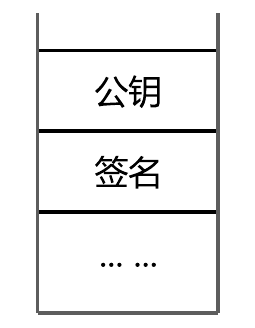OP_CHECKSIG 会先从栈中弹出公钥和签名，再根据该输入的 SIGHASH 标记计算交易摘要，然后完成 ECDSA 的验签

# 参考

]]>
<p>锁定脚本中的 OP_CHECKSIG 操作码，会要求解锁脚本能提供正确的 <a href="https://aaron67.cc/tags/ecdsa/">ECDSA</a> 签名。</p> <p>验证 ECDSA 签名是否有效，需要三个参数：公钥、签名和被签名的消息。对于 <a href="/2018/12/28/bitcoin-transaction-p2pk-p2pkh/" title="P2PKH">P2PKH</a> 这种最常见的使用了 OP_CHECKSIG 的交易模板：</p> <figure class="highlight plain"><table><tr><td class="code"><pre><span class="line">[签名] [公钥] OP_DUP OP_HASH160 [公钥哈希] OP_EQUALVERIFY OP_CHECKSIG</span><br></pre></td></tr></table></figure> <p>脚本中并没有<strong>直接提供</strong>被签名的消息的内容（<a href="/2018/12/28/bitcoin-transaction-p2pk-p2pkh/" title="P2PK">P2PK</a> 也是这样）。实际上，解锁脚本中的签名数据，由两部分构成：</p> <ul> <li>按 <a href="https://aaron67.cc/2020/10/10/bitcoin-sign-transaction/#%E5%BA%8F%E5%88%97%E5%8C%96-ECDSA-%E7%AD%BE%E5%90%8D">DER</a> 格式序列化后的 ECDSA 签名</li> <li>SIGHASH 标记</li> </ul> <p>在之前的<a href="https://aaron67.cc/2020/10/10/bitcoin-sign-transaction/#%E4%BA%A4%E6%98%93%E6%91%98%E8%A6%81">文章</a>中我们提过：“交易中被签名的消息，是交易本身。更准确的说，是通过 SIGHASH 标记区分的、交易中特定的数据子集”。交易本身在签名和验签时是已知的，也就是说，虽然脚本中没有直接提供消息的内容，但存储了能<strong>间接推算</strong>出消息内容的 SIGHASH 标记，它可以指示 OP_CHECKSIG 如何根据交易本身计算出这个消息（交易摘要），进而完成 ECDSA 的<a href="https://aaron67.cc/2020/09/30/ecdsa/#%E9%AA%8C%E8%AF%81">验签</a>。</p> <p>这篇文章，将介绍操作码 OP_CHECKSIG 的工作方式，以及 SIGHASH 标记的技术细节。</p>

# OP_BIN2NUM

• a 不要求最短编码（minimal encoding）。这点很好理解，如果必须是最短编码，那么 a 可以直接被解释成整数，不再需要额外的操作码
• x 是最短编码的，且长度不能超过 maxscriptnumlengthpolicy 的限制# OP_NUM2BIN

• a 不要求最短编码
• b 必须是最短编码，且长度不能超过 maxscriptnumlengthpolicy 的限制
• b 的值，不能小于 a 对应整数的最短编码的长度# 参考

]]>
<p>BSV 脚本提供了两个操作码，能让你“显式”的对栈上的字节序列和整数做“类型”转换。</p> <p>请在继续阅读前，先弄清楚 <a href="/2022/02/07/numbers-in-bsv-script/" title="BSV 脚本里整数的编码规则">BSV 脚本里整数的编码规则</a>。</p>
BSV 脚本里的整数 https://aaron67.cc/2022/02/07/numbers-in-bsv-script/ 2022-02-07T00:00:00.000Z 2023-01-25T09:43:22.863Z BSV 脚本里被送到栈上的数据，其数据类型都是字节序列（数组）。对于 OP_ADD 这类算术运算操作码，是如何将字节序列转成有符号整数的呢？

• 如果这个数据会被解释为整数，则使用带符号位的原码（signed magnitude）格式，编码成最短的小端字节序列
• 编码后的数据（字节序列）通过 pushdata 上栈

# 脚本中的整数编码

• 推送整数 0，必须使用 OP_0
• 推送其他整数，将该数按小端模式用原码格式编码成最短的字节序列（minimal encoding）后 pushdata（minimal push）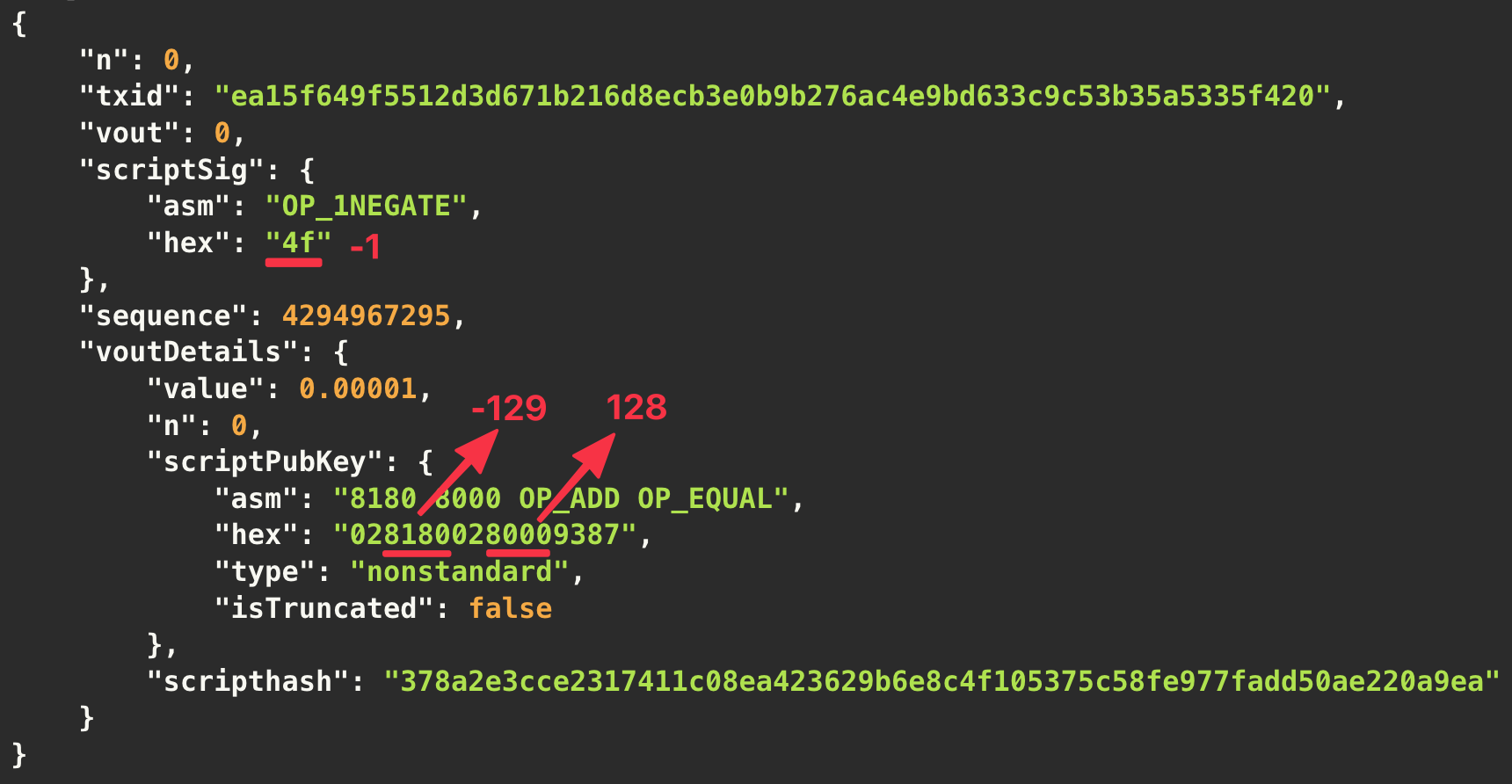# 参考

]]>
<p>BSV 脚本里被送到栈上的数据，其数据类型都是字节序列（数组）。对于 OP_ADD 这类算术运算操作码，是如何将字节序列转成有符号整数的呢？</p> <p>简单来说，只有两点：</p> <ul> <li>如果这个数据会被解释为整数，则使用带符号位的原码（signed magnitude）格式，编码成最短的小端字节序列</li> <li>编码后的数据（字节序列）通过 pushdata 上栈</li> </ul> <p>因为本质上还是使用 pushdata 并且这部分脚本会被执行，所以需要遵守<a href="/2022/02/06/pushdata-minimal-push-rule/" title="“最小推送”（minimal push）规则">“最小推送”（minimal push）规则</a>。</p>
Pushdata 的“最小推送”规则 https://aaron67.cc/2022/02/06/pushdata-minimal-push-rule/ 2022-02-06T00:00:00.000Z 2023-01-25T09:43:22.863Z 在 BSV 脚本中，将数据 0x01 送到栈上，似乎有两种做法：

1. 直接使用操作码 OP_1，对应的脚本为 { 0x51 }。根据定义，OP_1 会将 0x01 送到栈顶
2. 使用 pushdata 的方式，对应的脚本为 { 0x01 0x01 }。第一个 0x01 表示接下来的 1 字节数据（第二个 0x01）会被送到栈上

• 推送空的字节序列，必须使用操作码 OP_0，即 { 0x00 }
• 推送 0x01 ~ 0x10，必须使用操作码 OP_1 ~ OP_16，即 { 0x51 } ~ { 0x60 }
• 推送 0x81，必须使用操作码 OP_1NEGATE，即 { 0x4f }
• 推送其他数据，根据定义使用对应的 pushdata 操作码

]]>
<p>在 BSV 脚本中，将数据 0x01 送到栈上，似乎有两种做法：</p> <ol> <li>直接使用操作码 OP_1，对应的脚本为 { 0x51 }。根据定义，OP_1 会将 0x01 送到栈顶</li> <li>使用 <a href="https://wiki.bitcoinsv.io/index.php/Pushdata_Opcodes" target="_blank" rel="noopener">pushdata</a> 的方式，对应的脚本为 { 0x01 0x01 }。第一个 0x01 表示接下来的 1 字节数据（第二个 0x01）会被送到栈上</li> </ol> <p>实际上， 是错误的，因为它违反了“最小推送”（minimal push）规则。</p>

• 上海区块链开发者大会（2021.11）
• BSV 线上研讨会，比特币签名技术系列课程（2021.03）
• BSV 线上研讨会（2020.07）
]]>
<ul> <li>上海区块链开发者大会（2021.11）<ul> <li><a href="https://www.bilibili.com/video/BV14g411P7vR" target="_blank" rel="noopener">BSV 区块链基础设施软件及工具介绍
基于 Webot 2022 年总收入的权益 NFT 探索 https://aaron67.cc/2021/11/20/webot-2022-revenue-share-nft-exploration/ 2021-11-20T00:00:00.000Z 2023-01-25T09:43:22.864Z 大头鸟权益 NFT 的启发，我计划将 Webot 2022 年全年的 BSV 收入也映射成权益 NFT 自由认购。

这是一次全新的探索，如果你也对这样的实践感兴趣，请继续阅读。# 规则

Webot 一年的运行成本约 100 BSV，2022 年预计总收入 500 BSV。

所以会有 500 张权益 NFT 卡片被创造出来，每张卡片价值 1 BSV，对应全年总收入的千分之二分成。我自己保留 100 张卡片用于抵扣成本，不参与流通。

权益 NFT 的最终结算将在 2023 年 1 月 1 日进行。如果届时每张 NFT 的分红超过 1 BSV，那么你将根据实际情况获得收益。如果届时每张 NFT 的分红少于 1 BSV，那么你仍将获得 1 BSV。也就是说，这个 NFT 的权益是“保本”的。

目前，Webot 几乎所有的收入都来自用户发送的 MetaCoin 挖矿交易。所以如果 MetaCoin 能被持续赋能，或者 Webot 能在明年有比较大的用户和业务增长，你就很有可能因此而获得更多的 BSV。

# 获取方式

所有 400 张参与流通的 NFT 卡片

• 50 张仅限生态伙伴认购
• 100 张由活跃用户通过抢红包的方式提前认购
• 250 张通过 NFTOnShow 平台认购，北京时间 2021 年 12 月 3 日 21 时上线

# 卡片信息

专题页面

字段
Code Hash0d0fc08db6e27dc0263b594d6b203f55fb5282e2
Genesis ID4a4c589f5e53b5b0227b9de00e611c22ecebd2c2
Genesis TXIDcfed129fbea37525d87f16c2ec60c667a3247460d6834abf3f135c49c9c96192
Sensible ID9261c9c9495c133fbf4a83d6607424a367c660ecc2167fd82575a3be9f12edcf0000000

# 我为什么要做这件事

Webot 在没有任何宣传的情况下，能在四年多的时间里发展到今天这样的程度，已经远远超出了我的预期。盈利的多少并不是我持续维护项目的理由，要知道在之前的多年时间里 Webot 都没有任何收入。

如果明年 Webot 的全年收入超过 500 BSV，那么我只是少赚了点，如果不到 500 BSV，你跟我也都没有损失。

所以不管怎样，看起来都不算亏。整个实践可以被当成是一次“让利”推广，我希望 Webot 的用户和业务能不断增长，你在给朋友推荐时也会更有动力。

# 你的风险

请在参与活动之前认真理解规则，你可能损失 BSV 的场景包括但不限于

• 从其他人手中以过高的价格购买 NFT
• 我跑路了

# 审计

自北京时间 2022 年 1 月 1 日零时起至 2023 年 1 月 1 日零时止，Webot 将始终使用地址

收款，并定期合并 UTXO 以保证可用性，欢迎所有人监督。

# 结算

经过一年时间，截至 2023 年 1 月 1 日 0 时快照，共有 163 个地址持有卡片，Webot 2022 年的全年收入为 50.23797491 BSV。

根据约定，每张 NFT 卡片最终对应的权益为 1 BSV，结算交易已发

最后，感谢你的信任和参与。

]]>
<p>受<a href="https://www.nftonshow.com/collection/detail/DatoBird" target="_blank" rel="noopener">大头鸟权益</a> NFT 的启发，我计划将 <a href="https://webot.sv" target="_blank" rel="noopener">Webot</a> 2022 年全年的 BSV 收入也映射成权益 NFT 自由认购。</p> <p>这是一次全新的探索，如果你也对这样的实践感兴趣，请继续阅读。</p> <div style="width: 100%; margin: auto"><img src="https://aaron67-public.oss-cn-beijing.aliyuncs.com/202111212329327.JPG" alt></div>
徒手开二次根号 https://aaron67.cc/2021/10/13/calculate-square-root-with-pencil/ 2021-10-13T00:00:00.000Z 2023-01-25T09:43:22.862Z 抖音上看到的有趣解法（抖音号 huoxingketang）。只用纸笔，计算 $\sqrt{87}$ 的近似结果：

• 找到最大的整数使其平方小于 $87$，这个数是 9
• 计算 $87$ 与 $9^2$ 的差，结果为 6

$$\sqrt{87} \approx 9 + \cfrac{6}{2 \times 9} = 9\frac{1}{3} \approx 9.333333$$

用计算器算的结果 $\sqrt{87} \approx 9.32737905$。

这个解法用到的原理很简单。求 $\sqrt{x}$，设第一步找到的整数是 $a$，第二步算出的差为 $b$，则有

\begin{aligned}& x - a^2 = b \\[0.5em]\Rightarrow \quad & (\sqrt{x} + a)(\sqrt{x} - a) = b \\[0.5em]\Rightarrow \quad & \sqrt{x} - a = \frac{b}{\sqrt{x} + a} \\[0.5em]\Rightarrow \quad & \sqrt{x} = a + \frac{b}{a + \color{red}{\sqrt{x}}}\end{aligned}

其中 $\color{red}{\sqrt{x}}$ 可以被自己替换，搞出来连分式的形式：

$$\sqrt{x} = a + \cfrac{b}{a + a + \cfrac{b}{a + a + \cfrac{b}{a + a + \cdots}}} = a + \cfrac{b}{2a + \color{blue}{\cfrac{b}{2a + \cfrac{b}{2a + \cdots}}}}$$

注意到，我们选取的 $a$ 已经尽可能大了，这意味着 $b$ 会是一个比较小的数，所以省略上式中的蓝色部分，对整个分数值带来的误差会比较小，因为 $2a$ 比 $b$ 大的多，分数 $\cfrac{b}{2a}$ 是一个很小的数。例如

$$\cfrac{5}{72} \approx 0.069\color{red}{444}$$

$$\cfrac{5}{72 + \cfrac{5}{72}} = \cfrac{360}{5189} \approx 0.069\color{red}{378}$$

这就是在 $x$ 比较大时，$(a + \cfrac{b}{2a})$ 的值会非常接近 $\sqrt{x}$ 的原因。并且随着迭代次数的增多，结果的精度也会越高。

$$\sqrt{87} \approx 9 + \cfrac{6}{18 + \cfrac{6}{18}} = 9\frac{18}{55} \approx 9.327273$$

$$\sqrt{87} \approx 9 + \cfrac{6}{18 + \cfrac{6}{18 + \cfrac{6}{18}}} = 9\frac{55}{168} \approx 9.327381$$

$$\sqrt{87} \approx 9 + \cfrac{6}{18 + \cfrac{6}{18 + \cfrac{6}{18 + \cfrac{6}{18}}}} = 9\frac{1008}{3079} \approx 9.327379$$

$$\sqrt{87} \approx 9.32737905$$

注意到，对于像 $\sqrt{7}$ 这样比较小的数，运用此方法时需要多计算几层以保证准确，因为此时 $b$ 跟 $2a$ 的差距没有那么大，省略分母中的 $\cfrac{b}{2a}$ 会给结果带来较大误差。

$$\sqrt{7} \approx 2 + \cfrac{3}{4 + \cfrac{3}{4}} = 2\frac{12}{19} \approx 2.631579$$

$$\sqrt{7} \approx 2 + \cfrac{3}{4 + \cfrac{3}{4 + \cfrac{3}{4 + \cfrac{3}{4}}}} = 2\frac{264}{409} \approx 2.645477$$

或者更简单些，直接对 700 开方，再将得到的结果缩小 10 倍。

\begin{aligned}& \sqrt{700} \approx 26 + \cfrac{24}{52 + \cfrac{24}{52}} = 26\frac{156}{341} \approx 26.457478 \\[0.5em]\Rightarrow \quad & \sqrt{7} \approx 2.645748\end{aligned}

用计算器算的结果 $\sqrt{7} \approx 2.64575131$。

]]>
<p>抖音上看到的有趣解法（抖音号 huoxingketang）。只用纸笔，计算 $\sqrt{87}$ 的近似结果：</p> <ul> <li>找到最大的整数使其平方小于 $87$，这个数是 9</li> <li>计算 $87$ 与 $9^2$ 的差，结果为 6</li> </ul> <p>则</p> <p>$$<br>\sqrt{87} \approx 9 + \cfrac{6}{2 \times 9} = 9\frac{1}{3} \approx 9.333333<br>$$</p> <p>用计算器算的结果 $\sqrt{87} \approx 9.32737905$。</p>
Rabin 数字签名 https://aaron67.cc/2021/07/10/rabin-signatures/ 2021-07-10T00:00:00.000Z 2023-01-25T09:43:22.864Z 本文将详细介绍 Rabin 数字签名的算法细节。在开始之前，你需要对模运算、同余和模逆元的概念有一些了解。

# 算法过程

质数 $p$ 和 $q$，且有 $p \equiv 3 \pmod{4}$，$q \equiv 3 \pmod{4}$。

组合 $(p, q)$ 为私钥，两数的乘积 $n = pq$ 为对应的公钥。

使用私钥 $(p, q)$ 对消息 $m$ 签名的结果为组合 $(S, U)$，满足

$$S^2 \equiv H(m||U) \pmod{n} \tag{1}$$

其中的 $U$ 被称为填充值，$H(m||U)$ 的意思是将 $m$ 和 $U$ 的二进制流拼接在一起后再计算哈希，并将哈希结果（二进制流）看成一个整数。

令 $H = H(m||U) \bmod{n}$ 来简化书写。当 $U$ 确定后，可以用下式计算 $S$ 的值。

$$S = \left[q \cdot (H^{\frac{p+1}{4}} \bmod{p}) \cdot (q^{p-2} \bmod{p}) + p \cdot (H^{\frac{q+1}{4}} \bmod{q}) \cdot (p^{q-2} \bmod{q})\right] \bmod{n} \tag{2}$$

这样便得到了一组 $(S, U)$，将结果代入 (1) 式验算，若成立则返回这组结果，反之则改变 $U$ 的值重新计算 $S$，直到找到一组满足 (1) 式的解。

请注意，当 $p \equiv 3 \pmod{4}$ 时，$\frac{p+1}{4}$ 是一个整数，同理 $\frac{q+1}{4}$ 也是一个整数。

完整代码请参考 rabin.py。签名时，通过循环在 $m$ 后追加字节 0x00 来改变 $H$ 的值，并在计算出 $S$ 后验证 (1) 式是否成立，是则跳出循环返回，否则继续寻找。

验签时只需判断 (1) 式是否成立即可。

通过上面的描述你能看到，Rabin 签名的计算过程相对复杂，但验证过程极其简单。这个特性使得它非常适合在链上直接验签，虽然我们也可以在比特币脚本中实现 ECDSA 的验签逻辑，但它的计算成本要比验证 Rabin 签名高出许多。

整个算法设计基于这样的数学难题：在模是大合数的情况下，求二次剩余平方根是困难的。使用 Rabin 签名时，为了获得足够的安全性，公钥 $n$ 和哈希 $H(m||U)$ 的结果至少需要 3072 位（bit）。

至此，我们介绍了如何计算和验证 Rabin 签名，并给出了完整代码，方便你直接使用。如果你还关心这些结论和公式背后的推导过程，可以继续阅读，我们留了一些问题没有解答：

• 问题一：当 $p \equiv 3 \pmod{4}$ 时，为什么 $\frac{p+1}{4}$ 是一个整数？
• 问题二：公式 (1) 是如何推导出公式 (2) 的？

# 问题一

证明当 $p \equiv 3 \pmod{4}$ 时，$\frac{p+1}{4}$ 是一个整数。

根据同余的定义，对 $p \equiv 3 \pmod{4}$，存在整数 $m$、$n$ 和 $r$ 满足：

$$\begin{cases}p = 4m + r \\[0.5em]3 = 4n + r\end{cases}$$

两式相减，有

$$p - 3 = 4(m - n) \tag{3}$$

因为 $m$ 和 $n$ 都是整数，所以 $(m - n)$ 也是整数。也就是说，若两数在某个模数下同余，则两数的差是模数的整数倍，反之亦然

等式 (3) 两边同时加 4，得到 $p + 1 = 4(m - n + 1)$，即 $\frac{p+1}{4} = m - n + 1$。因为 $m$ 和 $n$ 都是整数，所以 $(m - n + 1)$ 也是整数，证毕。

# 二次剩余

在数论特别是同余理论中，一个整数 $X$ 对另一个整数 $p$ 的二次剩余（quadratic residue），指的是 $X$ 的平方 $X^2$ 除以 $p$ 得到的余数。

若存在某个 $X$ 使得 $X^2 \equiv d \pmod{p}$ 成立，则称 $d$ 是模 $p$ 的二次剩余。若对任意的 $X$ 式子 $X^2 \equiv d \pmod{p}$ 均不成立，则称 $d$ 是模 $p$ 的非二次剩余

例如，2 是模 7 的二次剩余，因为当 $X = 3$ 时 $3^2 \equiv 2 \pmod{7}$ 成立。3 是模 7 的非二次剩余，因为你找不到整数 $X$ 使式子 $X^2 \equiv 3 \pmod{7}$ 成立。

求二次剩余的平方根，即已知 $d$ 和 $p$ 求 $X$，可以使用 Tonelli–Shanks 算法。

当 $p \equiv 3 \pmod{4}$ 时，该算法有简化版本，即

$$X =\begin{cases}d^{\frac{p+1}{4}} \bmod{p} \\[0.5em]-d^{\frac{p+1}{4}} \bmod{p}\end{cases}$$

例如，当 $p = 7$ 时，若 $X^2 \equiv 2 \pmod{7}$，则

$$X =\begin{cases}2^{\frac{7+1}{4}} \bmod{7} = 4 \bmod{7} = 4 \\[0.5em]-2^{\frac{7+1}{4}} \bmod{7} = -4 \bmod{7} = 3\end{cases}$$

你可以验算一下，发现 $4^2 \bmod{7} = 2$，$3^2 \bmod{7} = 2$，根据公式计算出的 $X$ 是正确的结果。

如果你使用同样的方法，计算 $X^2 \equiv 3 \pmod{7}$，可以得到

$$X =\begin{cases}3^{\frac{7+1}{4}} \bmod{7} = 9 \bmod{7} = 2 \\[0.5em]-3^{\frac{7+1}{4}} \bmod{7} = -9 \bmod{7} = 5\end{cases}$$

再验算一下，发现 $2^2 \bmod{7} = 4 \neq 3$，$5^2 \bmod{7} = 4 \neq 3$，这两个解都是错误的。他们当然是错误的，因为 3 是模 7 的非二次剩余，没有这样的 $X$ 能满足方程。

所以在不确定 $d$ 是否是模 $p$ 的二次剩余时，用公式计算出结果后还需要验算。这也是在利用 (2) 式求解 $S$ 后还需要验算 (1) 式是否成立的原因，因为我们不确定 $H$ 是否是模 $n$ 的二次剩余。先记下这个问题：

• 问题三：在已知 $p$、$q$ 的情况下，有什么方法能快速判断 $H$ 是否是模 $n = pq$ 的二次剩余？

# 中国剩余定理

南北朝时期的数学著作《孙子算经》中提出了下列“物不知数”问题：

有物不知其数，三三数之剩二，五五数之剩三，七七数之剩二。问物几何？

即求解同时满足除以 3 余 2，除以 5 余 3，除以 7 余 2 的整数。中国剩余定理（也被称为中国余数定理和孙子定理）给出了此类问题有解的判定条件，并用构造法给出了在有解情况下解的具体形式。

对于 $x$ 的一元线性同余方程组：

$$\begin{cases}x \equiv a_1 \pmod{m_1} \\[0.5em]x \equiv a_2 \pmod{m_2} \\[0.5em]\quad... \\[0.5em]x \equiv a_n \pmod{m_n}\end{cases}$$

若整数 $m_1, m_2, …, m_n$ 任意两数互质，则对任意的整数 $a_1, a_2, …, a_n$，上述方程有解。通解可以用下面的步骤构造得到。

• 设 $M = m_1 \times m_2 \times … \times m_n$，$M_i = M / m_i$。即 $M_i$ 是除了 $m_i$ 以外的 $(n-1)$ 个整数的乘积
• 设 $t_i$ 是 $M_i$ 关于模 $m_i$ 的模逆元。即 $t_iM_i \equiv 1 \pmod{m_i}$
• 通解为 $x = kM + \sum_{i=1}^{n}a_it_iM_i$，其中 $k$ 是整数（$k \in \mathbb{Z}$）。即 $x \equiv \sum_{i=1}^{n}a_it_iM_i \pmod{M}$，最小整数解是 $(\sum_{i=1}^{n}a_it_iM_i) \bmod{M}$以求解“物不知数”问题为例。根据描述可以列出下列方程：

$$\begin{cases}x \equiv 2 \pmod{3} \\[0.5em]x \equiv 3 \pmod{5} \\[0.5em]x \equiv 2 \pmod{7}\end{cases}$$

对照着通解公式，计算过程为：

• $a_1 = 2$，$a_2 = 3$，$a_3 = 2$
• $m_1 = 3$，$m_2 = 5$，$m_3 = 7$
• $M = m_1m_2m_3 = 105$
• $M_1 = m_2m_3 = 35$，$M_2 = m_1m_3 = 21$，$M_3 = m_1m_2 = 15$
• $t_1 = M_1^{-1} \bmod{m_1} = 35^{-1} \bmod{3} = 2$，$t_2 = 21^{-1} \bmod{5} = 1$，$t_3 = 15^{-1} \bmod{7} = 1$
• $(\sum_{i=1}^{n}a_it_iM_i) \bmod{M}= (140 + 63 + 30) \bmod{105} = 233 \bmod{105} = 23$
• $x \equiv 23 \pmod{105}$

满足条件的解为 $23 + 105k$（$k \in \mathbb{Z}$），最小的整数解为 23。

# 问题二

有了二次剩余和中国剩余定理的知识基础，下面解答公式 (2) 是怎么来的。

根据 Rabin 签名的定义，有

$$S^2 \equiv H(m||U) \pmod{n} \tag{1}$$

其中 $n = pq$。注意到，$H(m||U)$ 可能不小于 $n$，令 $H = H(m||U) \bmod{n}$ 来简化书写，有

$$S^2 \equiv H \pmod{pq}$$

利用证明问题一时得到的推论，有 $S^2 - H = k \cdot pq = kq \cdot p = kp \cdot q$，其中的 $k$ 为整数（$k \in \mathbb{Z}$）。即两数的差是 $p$ 的整数倍，也是 $q$ 的整数倍，所以有

$$S^2 \equiv H \pmod{p} \tag{A}$$

$$S^2 \equiv H \pmod{q} \tag{B}$$

对 (A) 式使用 Tonelli–Shanks 算法的简化版本，可以得到

$$S =\begin{cases}H^{\frac{p+1}{4}} \bmod{p} \\[0.5em]-H^{\frac{p+1}{4}} \bmod{p} \tag{C}\end{cases}$$

同理对 (B) 式，也有

$$S =\begin{cases}H^{\frac{q+1}{4}} \bmod{q} \\[0.5em]-H^{\frac{q+1}{4}} \bmod{q} \tag{D}\end{cases}$$

为了使用中国剩余定理，需要保证整数 $m_1, m_2, …, m_n$ 任意两数互质。注意到 $p$ 和 $q$ 都是质数，所以 $p$ 和 $q$ 互质，我们从 (C) 式和 (D) 式中各挑一个解，组成下列方程：

$$\begin{cases}S = H^{\frac{p+1}{4}} \bmod{p} \\[0.5em]S = H^{\frac{q+1}{4}} \bmod{q} \tag{E}\end{cases}$$

则该方程有解。

• $a_1 = H^{\frac{p+1}{4}}$，$a_2 = H^{\frac{q+1}{4}}$
• $m_1 = p$，$m_2 = q$
• $M = pq = n$，$M_1 = q$，$M_2 = p$
• $t_1 = M_1^{-1} \bmod{m_1} = q^{-1} \bmod{p}$，$t_2 = M_2^{-1} \bmod{m_2} = p^{-1} \bmod{q}$

$$S = (\sum_{i=1}^{n}a_it_iM_i) \bmod{M}= \left[q \cdot H^{\frac{p+1}{4}} \cdot (q^{-1} \bmod{p}) + p \cdot H^{\frac{q+1}{4}} \cdot (p^{-1} \bmod{q})\right] \bmod{n} \tag{F}$$

如果你还记得费马小定理。对整数 $a$ 和质数 $p$，有 $a^p \equiv a \pmod{p}$，当 $a$ 不是 $p$ 的整数倍时，可以直接写成 $a^{p-1} \equiv 1 \pmod{p}$。你可以把这个等式理解成 $a \cdot a^{p-2} \equiv 1 \pmod{p}$，这正是模逆元的定义（一种求解模逆元的方法）。注意到 $p$ 和 $q$ 都是质数，所以 $p$ 不是 $q$ 的整数倍，$q$ 也不是 $p$ 的整数倍。所以有

$$p^{-1} \bmod{q} = p^{q-2} \bmod{q}$$

$$q^{-1} \bmod{p} = q^{p-2} \bmod{p}$$

代入 (F) 式，得到

$$S = \left[q \cdot H^{\frac{p+1}{4}} \cdot (q^{p-2} \bmod{p}) + p \cdot H^{\frac{q+1}{4}} \cdot (p^{q-2} \bmod{q})\right] \bmod{n} \tag{G}$$

为了获得足够的算法安全性，实际使用 Rabin 签名时选取的 $p$、$q$ 和 $H$ 会非常大，导致 (G) 式中的 $H^{\frac{p+1}{4}}$ 和 $H^{\frac{q+1}{4}}$ 部分无法计算。

注意到 (E) 式中的 $H^{\frac{p+1}{4}}$ 可能不小于 $p$，$H^{\frac{q+1}{4}}$ 可能不小于 $q$，所以 (E) 式实际上等价于

$$\begin{cases}S = (H^{\frac{p+1}{4}} \bmod{p}) \bmod{p} \\[0.5em]S = (H^{\frac{q+1}{4}} \bmod{q}) \bmod{q} \tag{E'}\end{cases}$$

观察方程 (E) 和公式 (G) 的形式，我们可以直接根据 (E’) 写出最终的 (2) 式。

$$S = \left[q \cdot (H^{\frac{p+1}{4}} \bmod{p}) \cdot (q^{p-2} \bmod{p}) + p \cdot (H^{\frac{q+1}{4}} \bmod{q}) \cdot (p^{q-2} \bmod{q})\right] \bmod{n} \tag{2}$$

其中的 $H = H(m||U) \bmod{n}$。虽然 $H^{\frac{p+1}{4}}$ 和 $H^{\frac{q+1}{4}}$ 无法求解，但计算 $(H^{\frac{p+1}{4}} \bmod{p})$ 和 $(H^{\frac{q+1}{4}} \bmod{q})$ 却很简单。

另外注意到，在构造方程 (E) 时，我们还有其他三种情况没有列出，所以 $S$ 的值还可能为

$$S = \quad\begin{cases}\quad \left[q \cdot (-H^{\frac{p+1}{4}} \bmod{p}) \cdot (q^{p-2} \bmod{p}) + p \cdot (H^{\frac{q+1}{4}} \bmod{q}) \cdot (p^{q-2} \bmod{q})\right] \bmod{n} \\[0.5em]\quad \left[q \cdot (H^{\frac{p+1}{4}} \bmod{p}) \cdot (q^{p-2} \bmod{p}) + p \cdot (-H^{\frac{q+1}{4}} \bmod{q}) \cdot (p^{q-2} \bmod{q})\right] \bmod{n} \\[0.5em]\quad \left[q \cdot (-H^{\frac{p+1}{4}} \bmod{p}) \cdot (q^{p-2} \bmod{p}) + p \cdot (-H^{\frac{q+1}{4}} \bmod{q}) \cdot (p^{q-2} \bmod{q})\right] \bmod{n}\end{cases}$$

运行代码 rabin.py 中的例子，可以得到签名 (71, 0x00)。改变 $S$ 的计算公式，你会发现 (27, 0x00)、(50, 0x00) 和 (6, 0x00) 都是合法的签名。如何计算 $-H^{\frac{p+1}{4}} \bmod{p}$ 和 $-H^{\frac{q+1}{4}} \bmod{q}$，留给你思考。

# 问题三

在计算签名时，因为不确定 $H$ 是否是模 $n$ 的二次剩余，所以在利用公式 (2) 计算出 $S$ 后，还需要代入 (1) 式验算，验算失败就要重做。如果我们可以在计算 $S$ 前就确定 $H$ 是模 $n$ 的二次剩余，那么可以减少签名过程中的一些无效运算。

数论中的欧拉准则（也叫欧拉判别法）可以用来确定给定的整数是否是一个质数的二次剩余。

若 $p$ 是奇质数（除了 2 以外的质数）且 $p$ 不能整除 $d$（即 $d$ 不是 $p$ 的整数倍），当且仅当

$$d^{\frac{p-1}{2}} \equiv 1 \pmod{p}$$

成立时，$d$ 是模 $p$ 的二次剩余。若 $p = 2$，或 $d$ 是 $p$ 的整数倍，则 $d$ 一定是模 $p$ 的二次剩余，无需判定。

Rabin 签名中的 $p$ 和 $q$ 都是奇质数，但 (1) 式中的模数 $n = pq$ 不是。下面我们来证明：对质数 $p$、$q$，若 $d$ 是模 $p$ 的二次剩余，也是模 $q$ 的二次剩余，那么 $d$ 也是模 $pq$ 的二次剩余

根据条件及二次剩余的定义，存在整数 $a$、$b$ 满足

$$\begin{cases}a^2 \equiv d \pmod{p} \\[0.5em]b^2 \equiv d \pmod{q}\end{cases}$$

根据中国剩余定理，因为 $p$、$q$ 互质，所以存在这样的整数 $x$ 满足

$$\begin{cases}x \equiv a \pmod{p} \\[0.5em]x \equiv b \pmod{q}\end{cases}$$

所以 $x^2 \bmod{p} = a^2 \bmod{p} = d \bmod{p}$，即 $x^2 \equiv d \pmod{p}$。也就是说，$(x^2 - d)$ 是 $p$ 的整数倍，即存在整数 $k$ 满足 $x^2 - d = kp$。

同理 $x^2 \bmod{q} = b^2 \bmod{q} = d \bmod{q}$，即 $x^2 \equiv d \pmod{q}$。也就是说，$(x^2 - d)$ 也是 $q$ 的整数倍，即 $kp$ 是 $q$ 的整数倍。

因为 $p$、$q$ 互质，所以 $p$ 不是 $q$ 的整数倍，所以 $k$ 是 $q$ 的整数倍。因此 $x^2 - d = kp$ 是 $pq$ 的整数倍，即 $x^2 \equiv d \pmod{pq}$，证毕。

有了这个结论，我们可以对代码做一些优化，改进后的代码请参考 rabin.py。先用欧拉准则确定 $H$ 是模 $p$ 的二次剩余且是模 $q$ 的二次剩余后，再计算 $S$。

# 致谢

虽然只是篇博客文章，但完成它于我并非易事。

特别感谢 nChain Research Team 的 Wei Zhang，对本文内容的审阅和优化，以及对我理解相关数学原理和密码学知识的指导和帮助。

特别感谢 nChain Research Team 的 Owen Vaughan，对我理解 Rabin 签名的指导和帮助。

感谢中国人民大学的研究生李小满，为文中部分证明提供思路。

感谢感应合约团队的顾露、陈诚、蒋杰和陈耀欢，ShowPay 的王宇，以及 sCrypt 的郑宏峰，对我在使用和实践 Rabin 签名过程中的指导和帮助。

谢谢你们在工作和生活之余挤出宝贵时间，热情而耐心的解答我的问题，无私的分享知识和经验。

# 参考

]]>
<p>本文将详细介绍 <a href="https://en.wikipedia.org/wiki/Rabin_signature_algorithm" target="_blank" rel="noopener">Rabin 数字签名</a>的算法细节。在开始之前，你需要对<a href="/2020/05/30/modular-multiplicative-inverse/" title="模运算、同余和模逆元">模运算、同余和模逆元</a>的概念有一些了解。</p>
我被盗了 600 BSV https://aaron67.cc/2020/11/08/lost-600-bsv/ 2020-11-08T00:00:00.000Z 2023-01-25T09:43:22.863Z 请立即停止使用 ElectrumSV 1.3.7 及之前版本中实现的 multisig accumulator 多签方案。该方案的锁定脚本有严重 bug，我被盗了 600 BSV。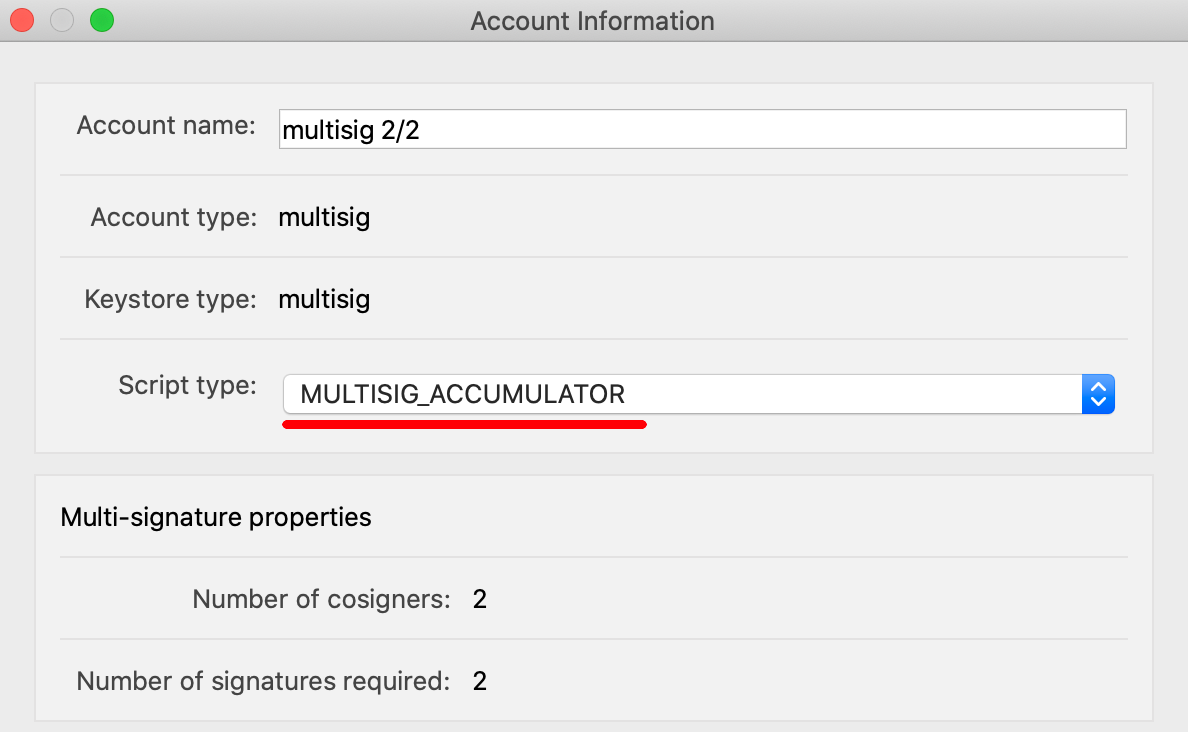# 背景

CoinGeek 2019 首尔大会时，介绍过 multisig accumulator 多签方案。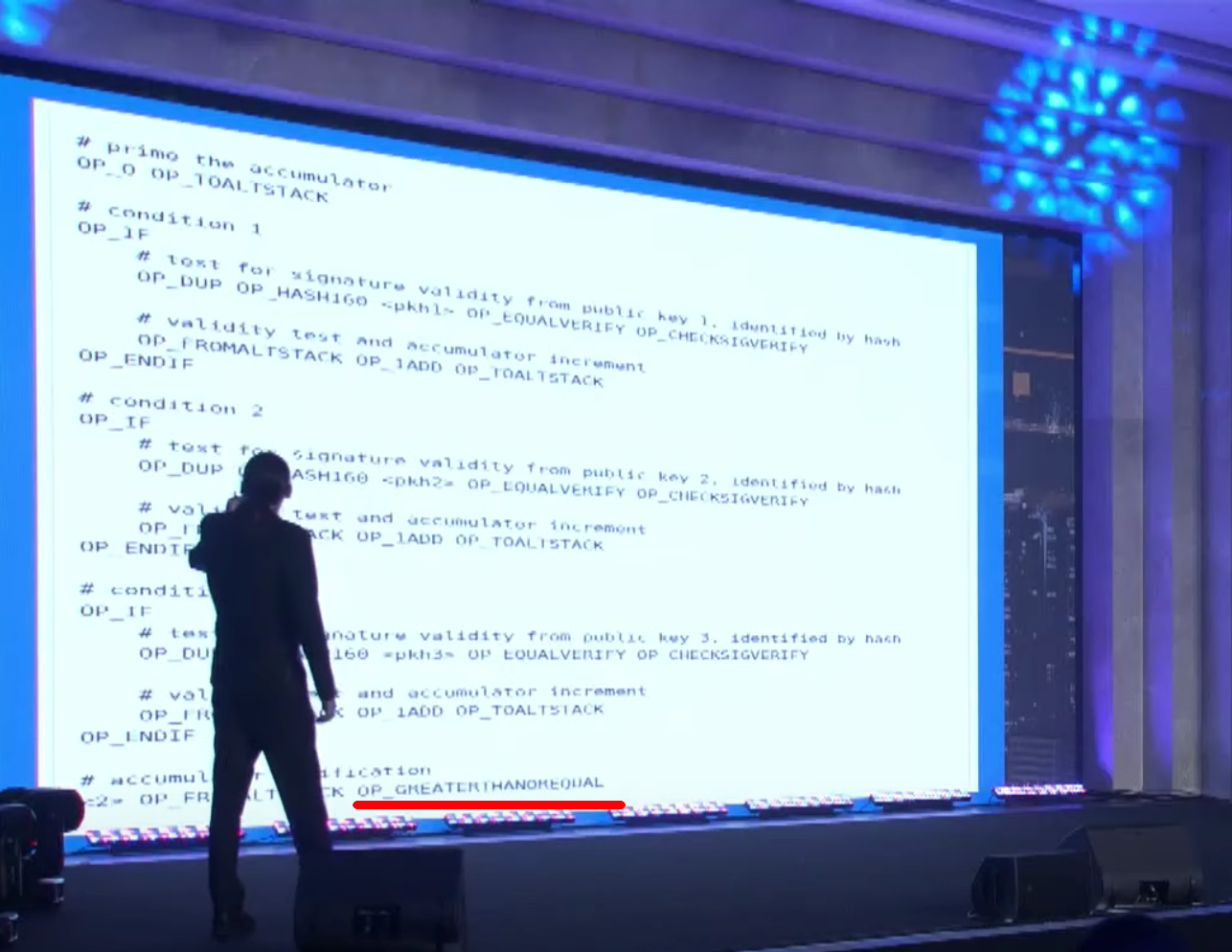ElectrumSV 在 1.3.0b4 版本中实现了该功能，源码如下。

# 大致经过

事情发生在 11 月 6 号。

1. 凌晨 2 点多，我输出了 6 个 multisig accumulator UTXO，每个 100 BSV。
2. 当天晚上 10 点半，我不太放心，又重新整理了一遍这 600 BSV，输出了 6 个新的 multisig accumulator UTXO，每个 100 BSV。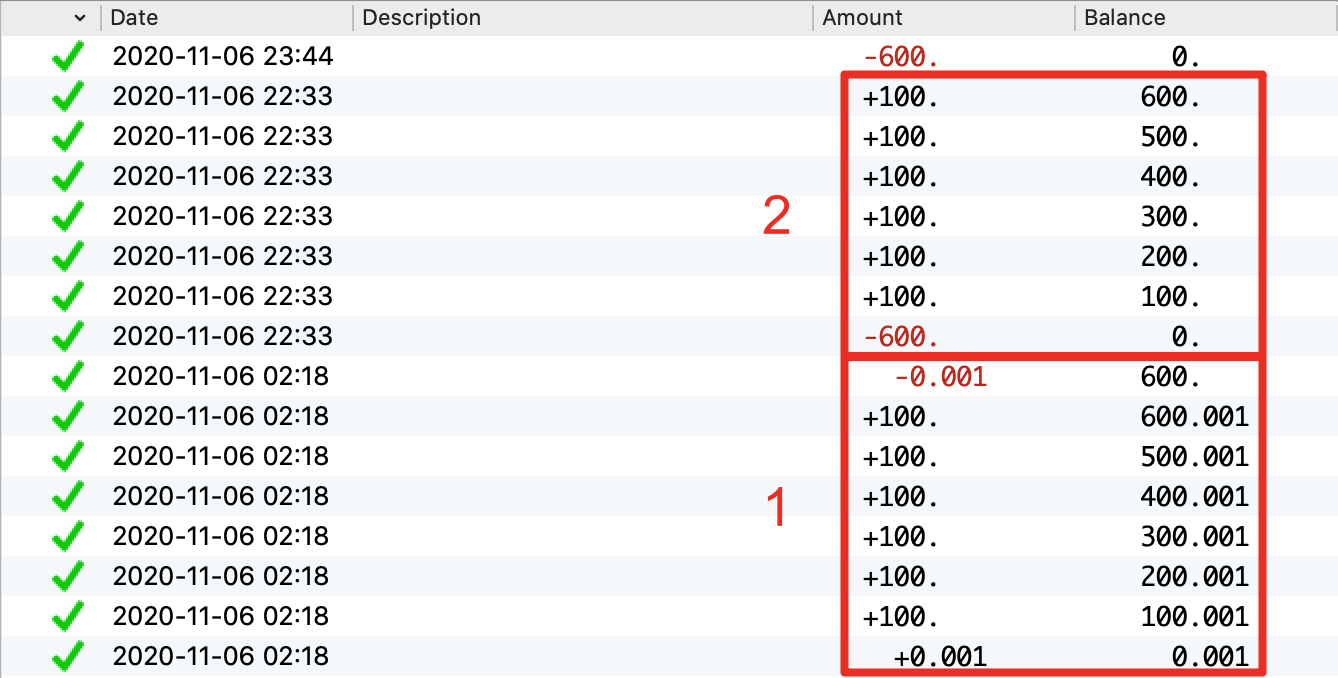从我第一次使用该方案大额存币，到第二次整理，之间间隔 20 小时，黑客都没有动作。

在我完成第二次操作一小时后，黑客利用漏洞将我的币全部转到了地址 1LcKTzSzpMAwH4bzymGSkbhY2EBpmT7n5J，对应的交易

第二天，7 号晚上，我发现被盗。

# 问题分析

输出到 accumulator 脚本的 UTXO，被下面这样的锁定脚本锁定。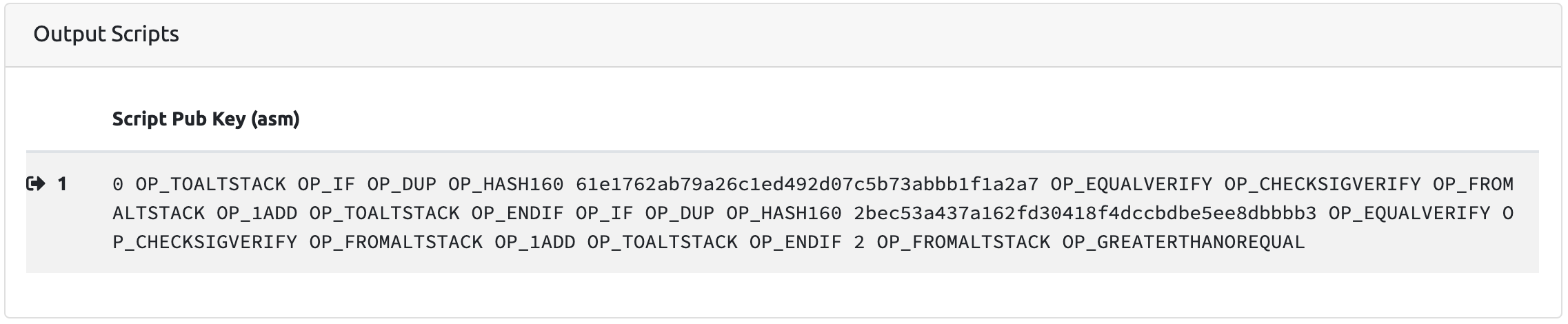按照设计，花费这个 UTXO 的解锁脚本中提供的签名个数，要不小于多签的阈值。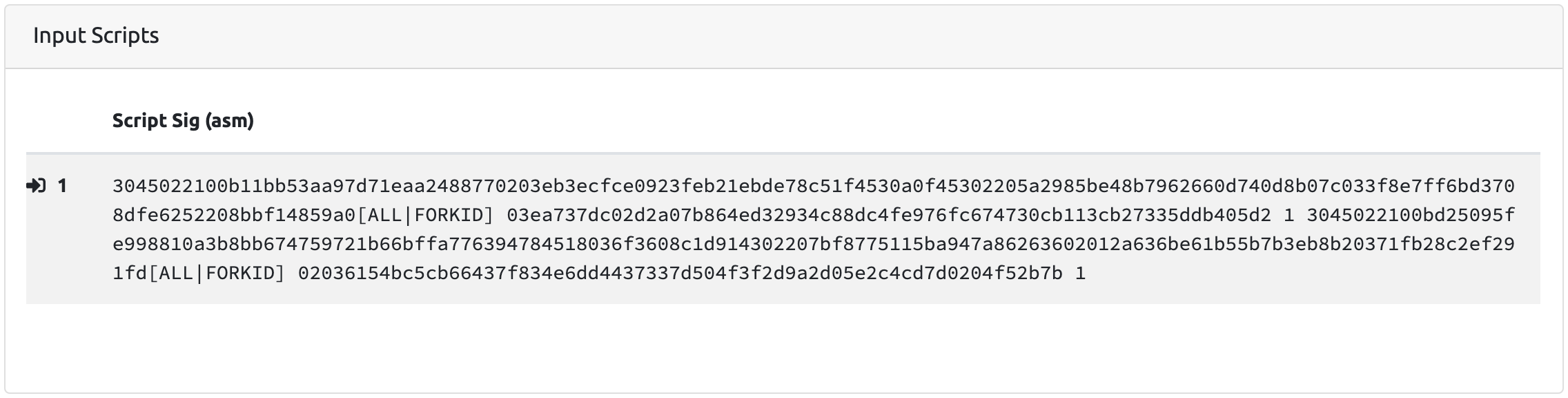下面这笔交易，盗走了我 600 BSV，使用的解锁脚本是“0 0”。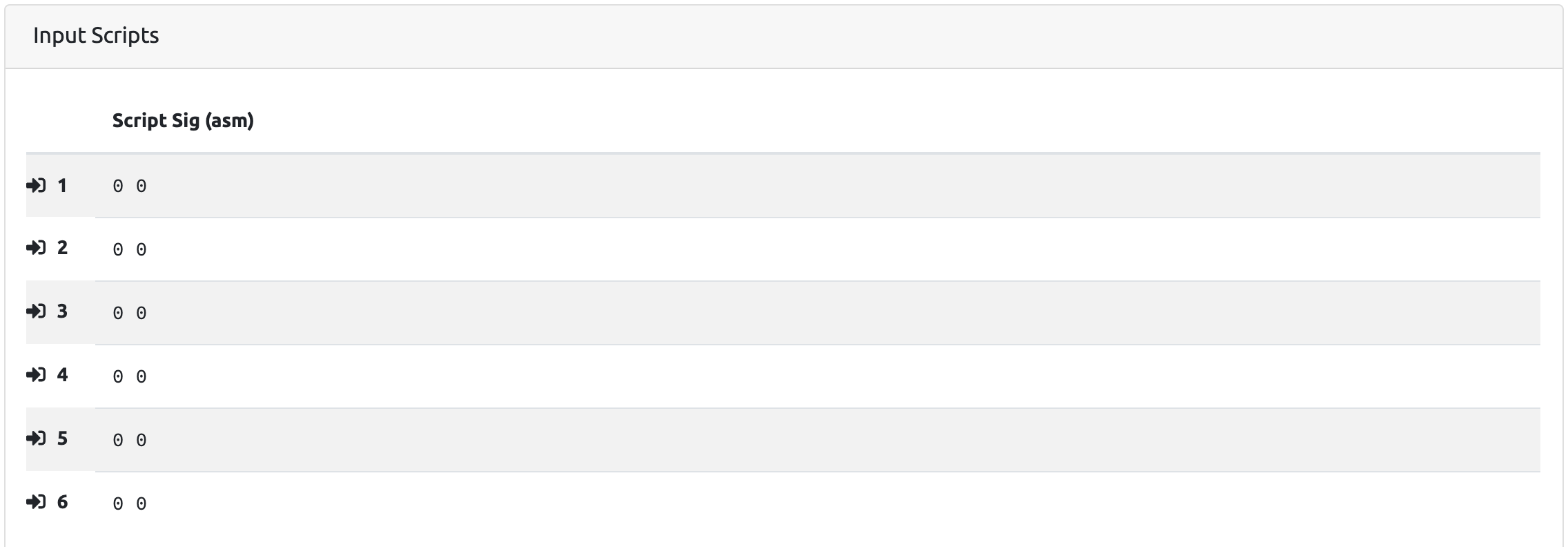问题出在最后比较 [需要的签名个数] 和 [解锁脚本中的签名个数] 时，使用了错误的操作码 OP_GREATERTHANOREQUAL。

这个操作码实际在验证 [需要的签名个数] 是否大于等于 [解锁脚本中的签名个数]。很明显，这是一个逻辑错误，应该判断是否小于等于。

详细的脚本执行过程，可以参考 Note.SV 作者李龍的分析文档

# 最后的话

事情发生后，我第一时间联系了 ElectrumSV 的作者 Roger Taylor，他随后确认了这个严重的 bug。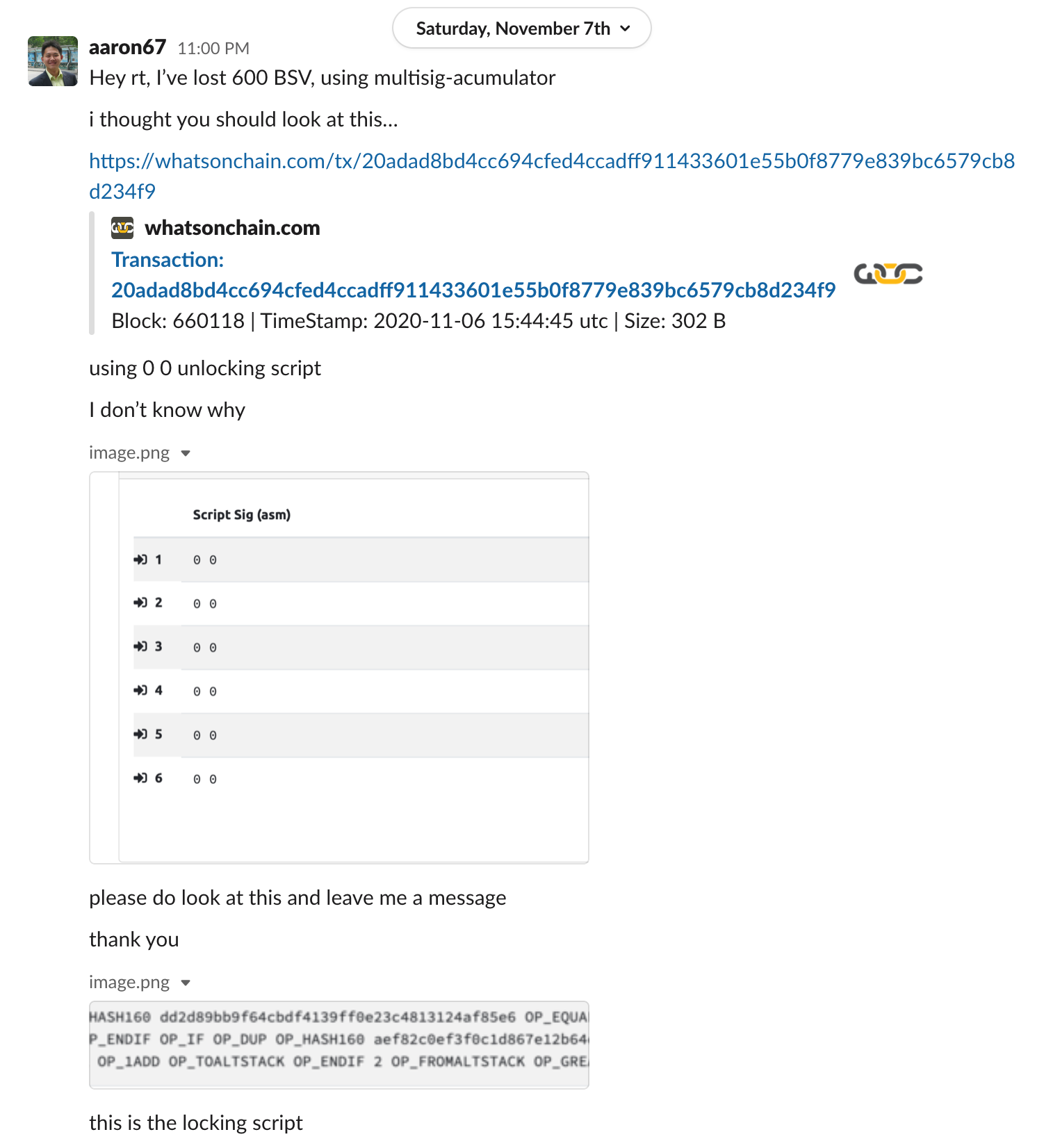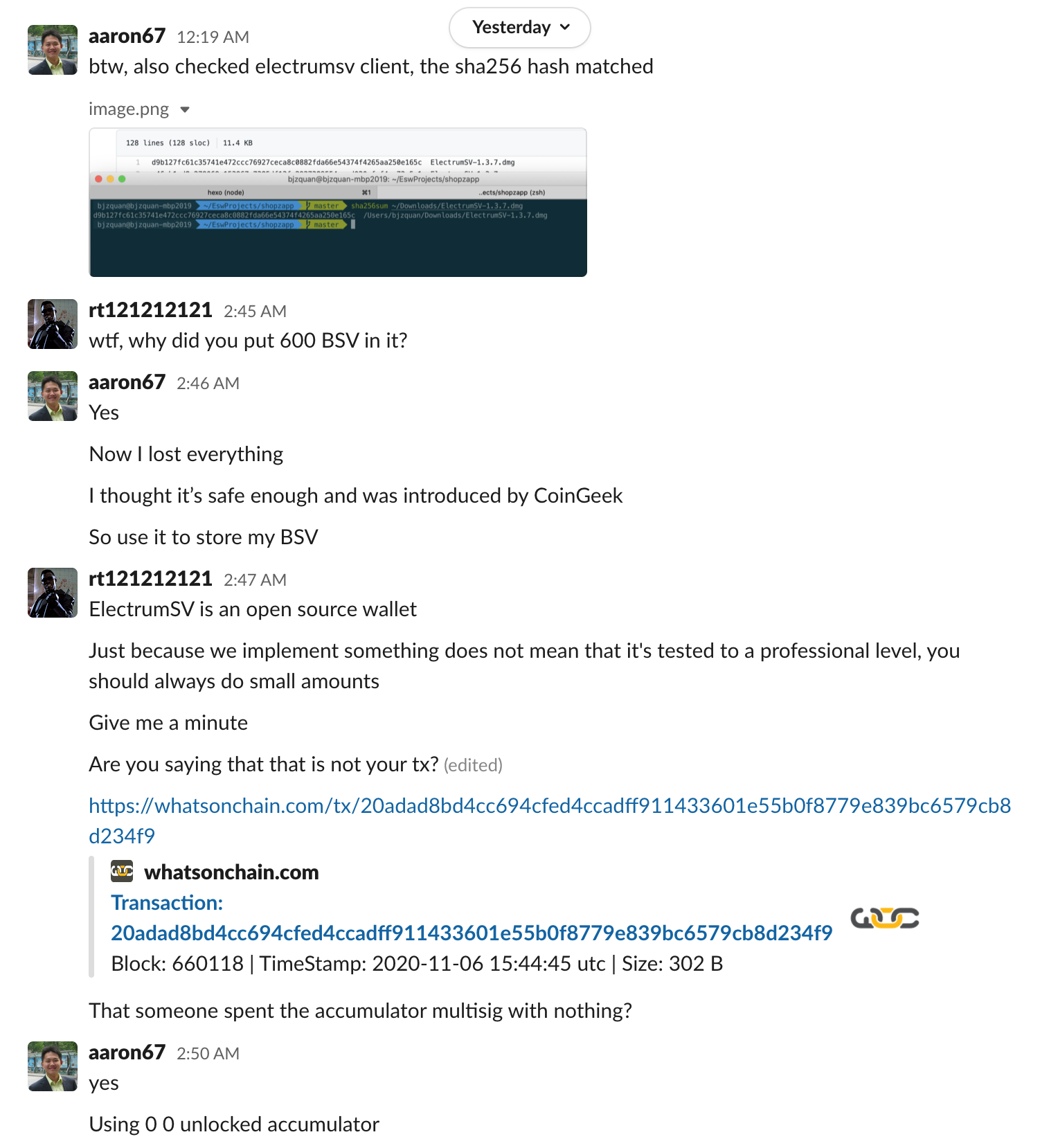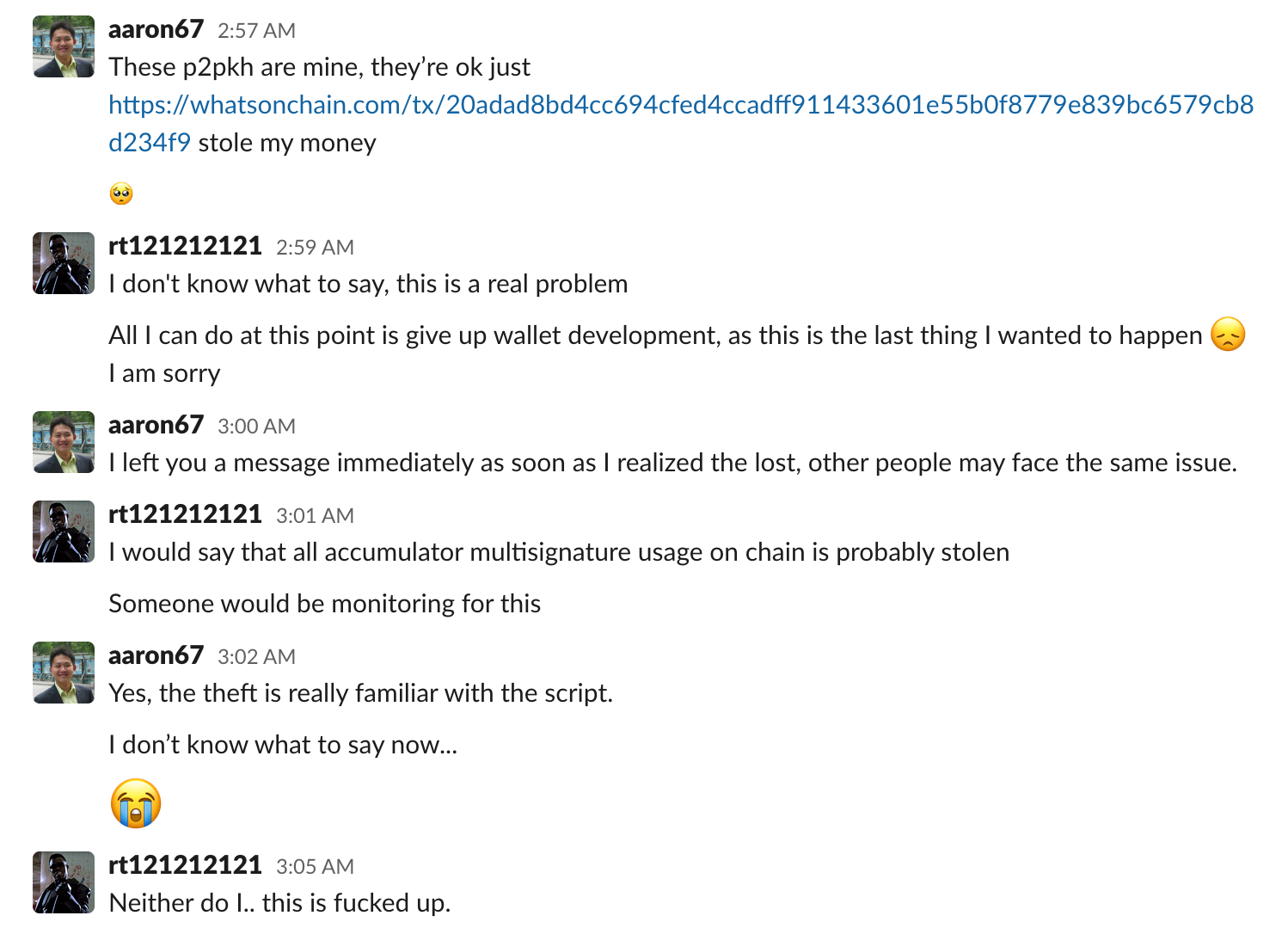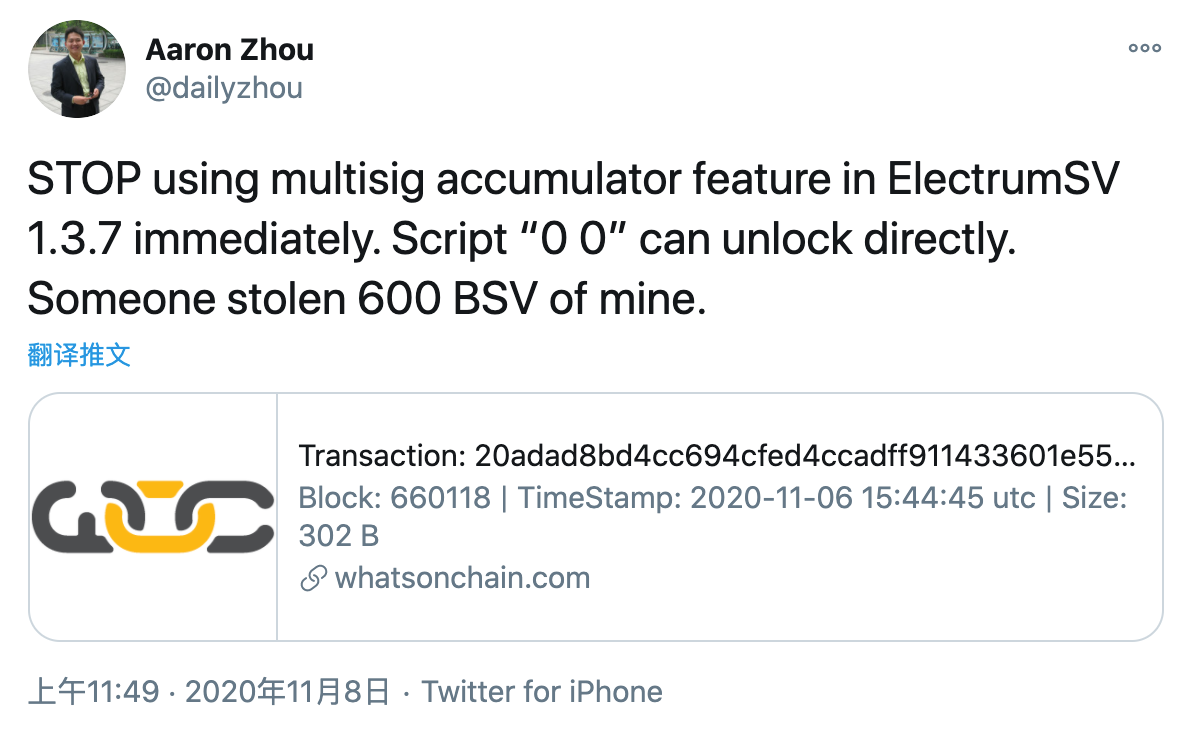需要澄清的是，丢币不是因为私钥泄露，也不是使用了有问题的 ElectrumSV 客户端，更不是因为 Bitcoin SV 网络有什么漏洞。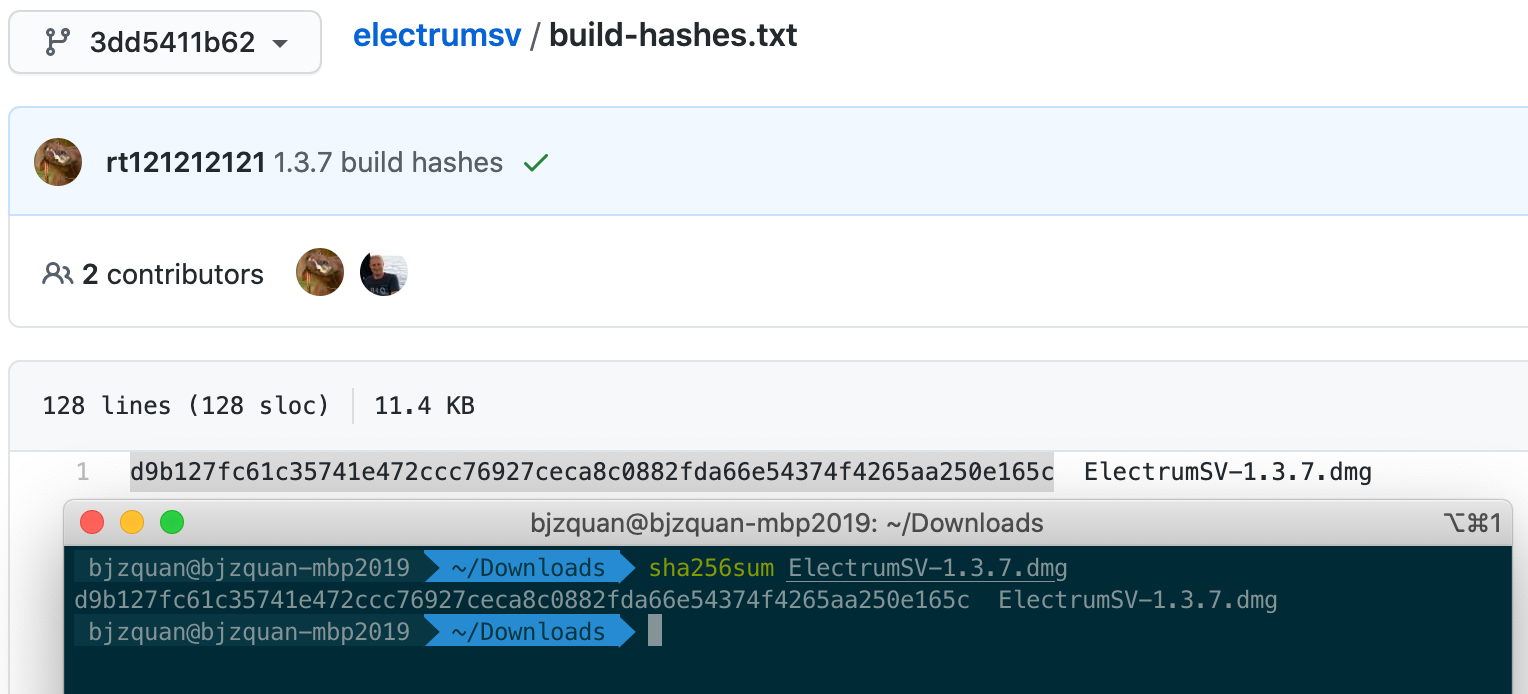P2PKH 脚本没有问题，传统多签方案 multisig bare 也没有问题，Electrum SV 钱包在其他方面的安全性不受影响。

多签方案 multisig accumulator 的 bug 出自理论原型，Roger 在实现时直接参考了演示代码，也没有做足够多的测试。而我对这样的新技术没有抵抗力，直接使用该方案大量存币，最终酿成悲剧。

最后，衷心感谢每位朋友的关心，经历这样的事情十分痛苦，但你们让我感到温暖。

]]>
<p>请立即停止使用 <a href="https://electrumsv.io/" target="_blank" rel="noopener">ElectrumSV</a> 1.3.7 及之前版本中实现的 multisig accumulator 多签方案。该方案的锁定脚本有严重 bug，我被盗了 600 BSV。</p> <div style="width: 50%; margin: auto"><img src="https://aaron67-public.oss-cn-beijing.aliyuncs.com/20201108224929.png" alt></div>
拉格朗日插值法 https://aaron67.cc/2020/10/29/lagrange-interpolate/ 2020-10-29T00:00:00.000Z 2023-01-25T09:43:22.863Z 给定 $(t+1)$ 个不同的点，过这些点且最高次不大于 $t$ 的多项式，有且只有一条。

本文将介绍如何使用拉格朗日插值法，求解这样的多项式。

# 方法

已知点 $(x_0, y_0)$、$(x_1, y_1)$、…、$(x_t, y_t)$，拉格朗日插值法的思路是寻找多项式 $l_j(x)$，使其在 $x = x_j$ 时的取值为 1，在其他点的取值都是 0。

这样的多项式很好构造。

$$l_j(x) = \frac{(x-x_0)…(x-x_{j-1})(x-x_{j+1})…(x-x_t)}{(x_j-x_0)…(x_j-x_{j-1})(x_j-x_{j+1})…(x_j-x_t)}$$

注意到，当 $x=x_j$ 时，$l_j(x_j)$ 的分子和分母相同，所以 $l_j(x_j)=1$，当 $x$ 取其他值时，其分子总是 0，所以结果为 0。

多项式 $l_j(x)$ 就像开关一样，可以让 $y_jl_j(x)$ 满足只有在 $x = x_j$ 时的取值为 $y_j$，而在其他点的取值都是 0。

基于此，可以继续构造多项式

$$L(x) = y_0l_0(x) + y_1l_1(x) + … + y_tl_t(x)$$

满足过已知的 $(t+1)$ 个点。给定点 $(x_0, y_0) = (1, 350)$、$(x_1, y_1) = (2, 770)$ 和 $(x_2, y_2) = (3, 1350)$，求过这三个点的多项式。

先构造 $l_j(x)$，有

$$l_0(x) = \frac{(x - x_1)(x - x_2)}{(x_0 - x_1)(x_0 - x_2)} = \frac{(x-2)(x-3)}{(1-2)(1-3)} = \frac{1}{2}x^2 - \frac{5}{2}x + 3$$

$$l_1(x) = \frac{(x-x_0)(x-x_2)}{(x_1-x_0)(x_1-x_2)} = \frac{(x-1)(x-3)}{(2-1)(2-3)} = -x^2 + 4x - 3$$

$$l_2(x) = \frac{(x-x_0)(x-x_1)}{(x_2-x_0)(x_2-x_1)} = \frac{(x-1)(x-2)}{(3-1)(3-2)} = \frac{1}{2}x^2 - \frac{3}{2}x + 1$$

再构造 $L(x)$，有

$$L(x) = \sum_{i=0}^{2}y_il_i(x) = 350(\frac{1}{2}x^2 - \frac{5}{2}x + 3) + 770(-x^2 + 4x - 3) + 1350(\frac{1}{2}x^2 - \frac{3}{2}x + 1) = 80x^2 + 180x + 90$$

求解完毕。

# 证明

## 存在性

显而易见，我们总能按公式构造出 $l_j(x)$，从而构造出 $L(x)$。

注意到，$l_j(x)$ 的分子是 $t$ 个 1 次多项式相乘，分母是一个整数，所以 $l_j(x)$ 是一个最高次不大于 $t$ 的多项式。

任意个最高次不大于 $t$ 的多项式相加，结果多项式的最高次也不会大于 $t$，所以 $L(x)$ 是一个最高次不大于 $t$ 的多项式。

## 唯一性

假设这样的多项式不唯一，对任意两个最高次不大于 $t$ 的拉格朗日多项式 $L_1$ 和 $L_2$。

因为 $L_1$ 和 $L_2$ 都过已知的 $(t+1)$ 个点，所以两者的差 $(L_1 - L_2)$ 在这 $(t+1)$ 个点的取值都是 0。

也就是说，$(L_1 - L_2)$ 一定是多项式 $(x-x_0)(x-x_1)…(x-x_t)$ 的倍数。

若 $L_1 - L_2 \neq 0$，则其次数一定不小于 $(t+1)$。

而任意个最高次不大于 $t$ 的多项式相减，结果多项式的最高次也不会大于 $t$，所以 $(L_1 - L_2)$ 的最高次也不大于 $t$。

矛盾。

所以 $L_1 - L_2 = 0$，即 $L_1 = L_2$。

# 完整代码

polynomial.py

Polynomial用来表示有限域 Secp256k1.n 上的多项式

$$y \equiv a_0x^0 + a_1x^1 + … a_tx^t \pmod{Secp256k1.n}$$

并实现了多项式加法、乘法和求值等基本运算。

对于方法interpolate_evaluate，我们的目标是计算插值得到的多项式在某点的取值，所以并不需要知道 $L(x)$ 的具体形式，可以直接将参数 $x$ 的值带入公式计算最终结果。方法的内层循环用来计算 $l_i(x)$ 的分子和分母的值，外层循环将每个 $y_il_i(x)$（分子和分母）的值保存到数组lagrange中，最后对所有的 $y_il_i(x)$ 通分并求和，得到 $L(x)$ 的值。

# 参考

]]>
<p>给定 $(t+1)$ 个不同的点，过这些点且最高次不大于 $t$ 的多项式，有且只有一条。</p> <p>本文将介绍如何使用拉格朗日插值法，求解这样的多项式。</p>
Bitcoin SV 交易的粉尘限制 https://aaron67.cc/2020/10/28/bitcoin-sv-dust/ 2020-10-28T00:00:00.000Z 2023-01-25T09:43:22.860Z 在第一届“打点创新营”上，我分享了有关 546 的故事。

对 Bitcoin SV 交易的 P2PKH 输出，如果其金额小于 546 聪，则该交易是粉尘交易（dust），不会被网络接受。

1.0.5 版本的节点开始，这个限制将变成 135 聪。

# 回顾

之前的文章中提到，交易输出的 dust 阈值由函数GetDustThreshold计算。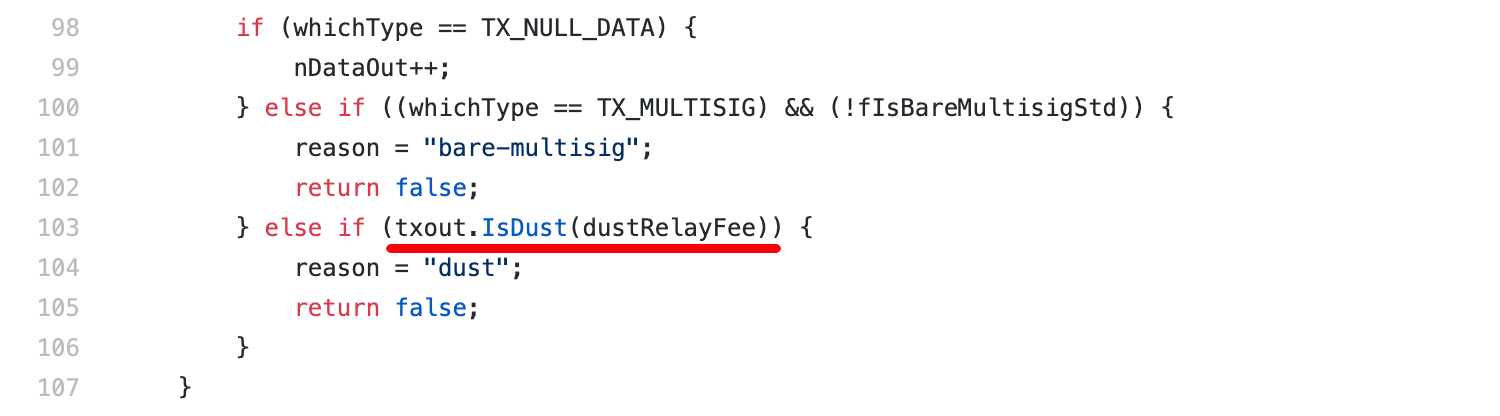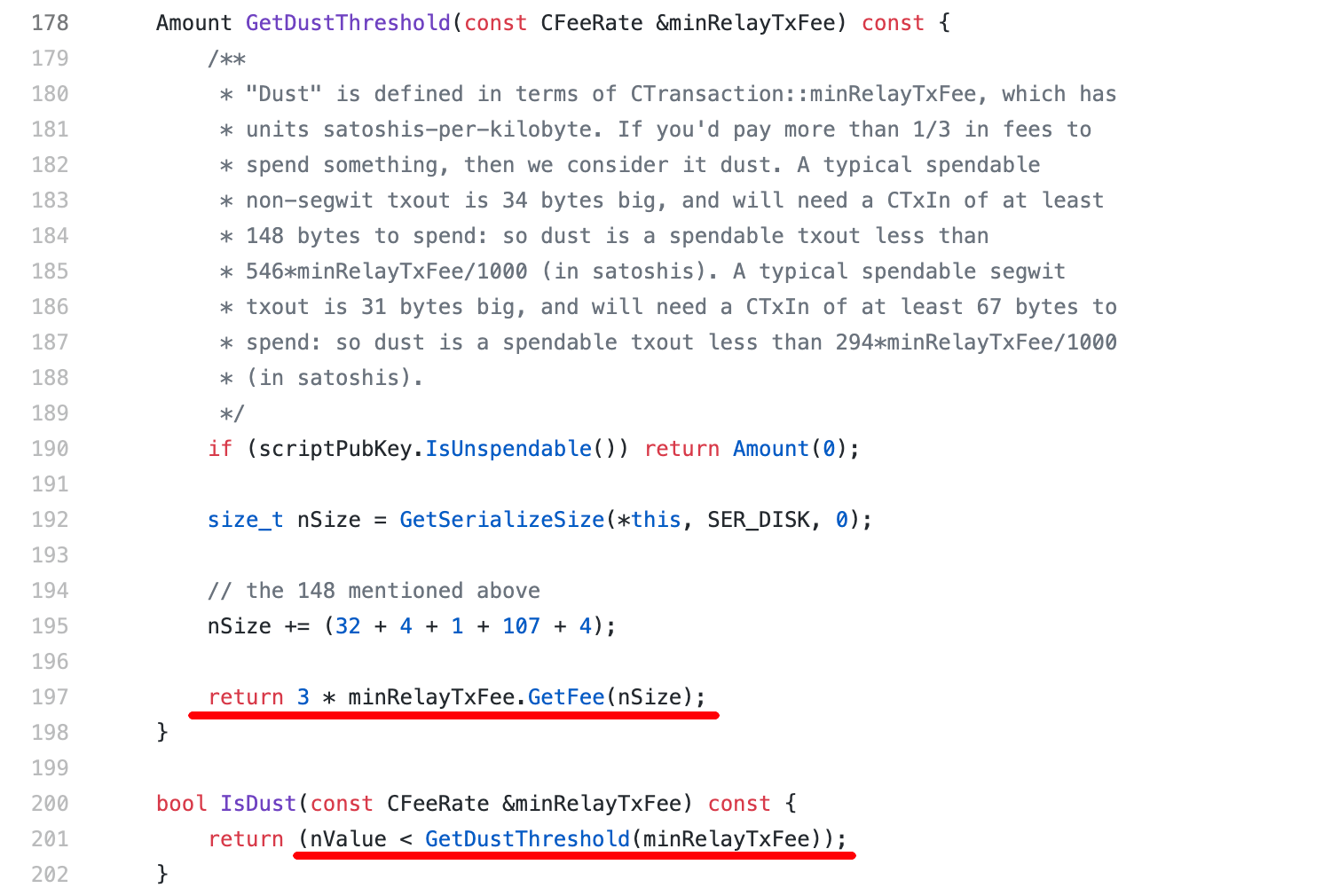根据定义，如果花费一个 UTXO 时需要支付的手续费超过了这个 UTXO 面值的 1/3，那么这个 UTXO 是 dust，生成该 UTXO 的交易不会被网络接受。

请注意调用IsDust时的实参dustRelayFee，找一下变量定义。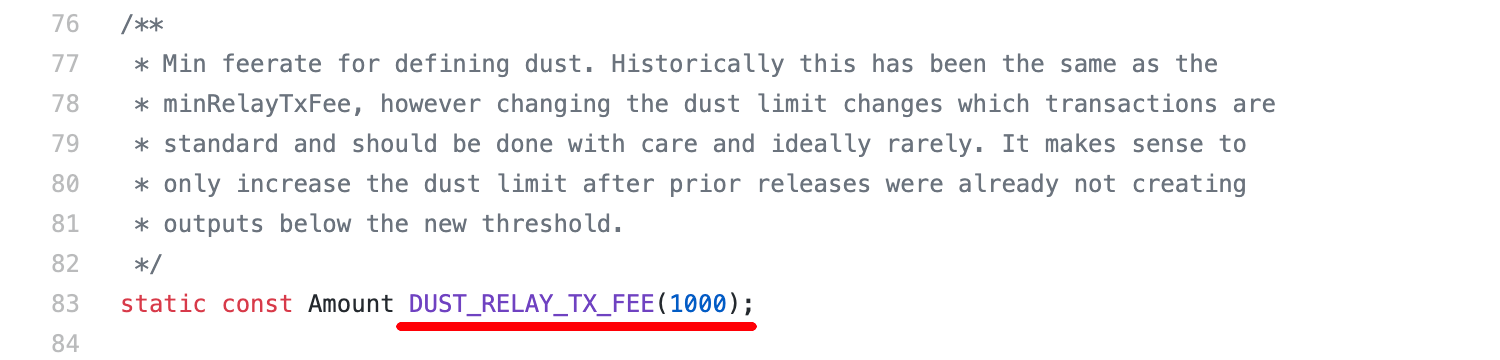花费一笔 P2PKH 的输出需要 182 字节，包括 148 字节的输入和 34 字节的输出。传入的费率是dustRelayFee，由DUST_RELAY_TX_FEE的值初始化为 1 聪/字节。所以最终计算出的 dust 阈值是 546 聪。

# 现状

创世纪升级调整了全网的默认交易费率。

DUST_RELAY_TX_FEE当时并未调整，仍为 1 聪/字节。这会造成困扰，因为从函数定义和变量命名来看，dust 阈值跟最小交易传播费率有关。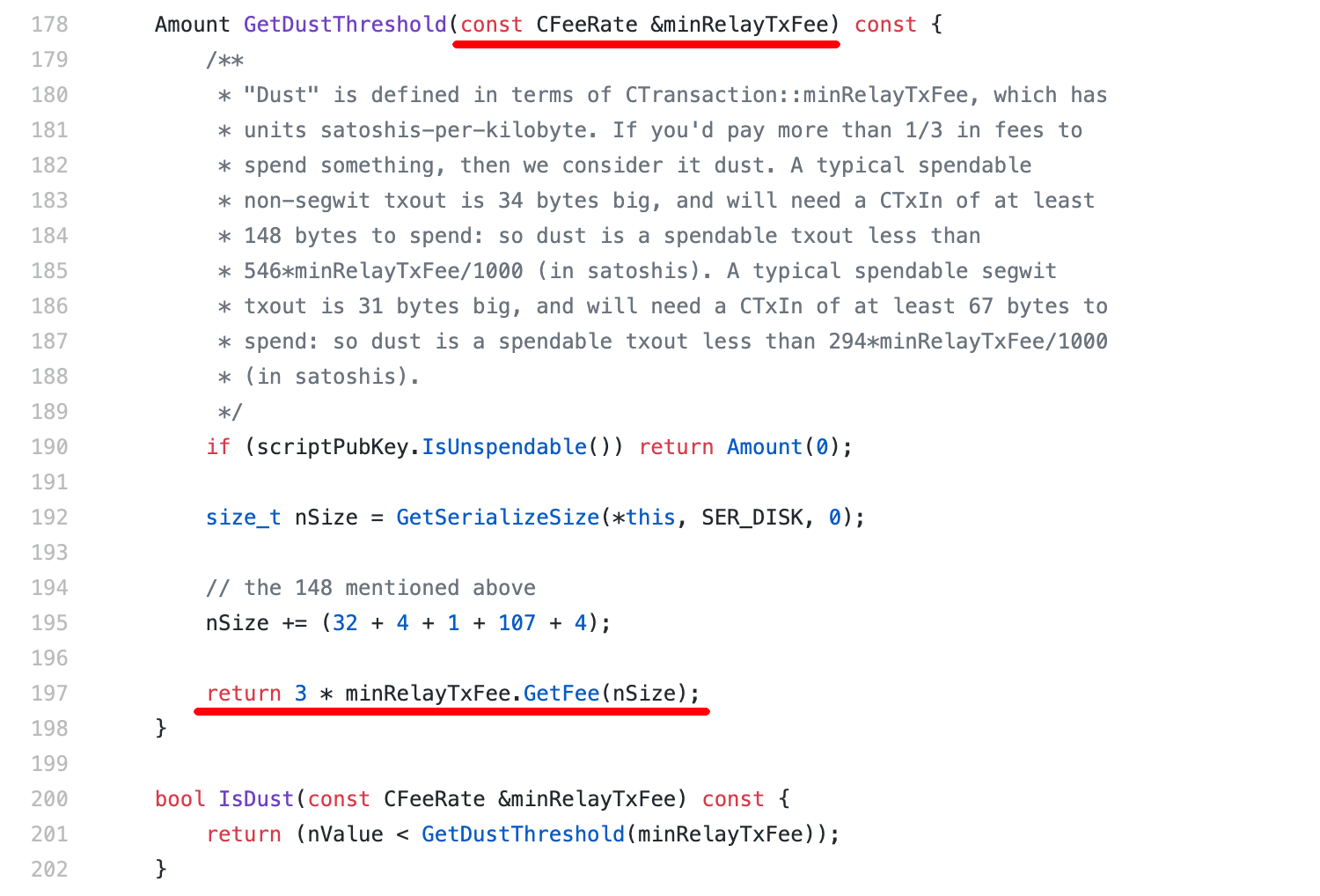查看 1.0.5 版本的提交记录，能找到今年 5 月的一次更新。新代码并未修改 dust 的计算逻辑，只是将DUST_RELAY_TX_FEE调整为 0.25 聪/字节，与默认的最小交易传播费率保持一致。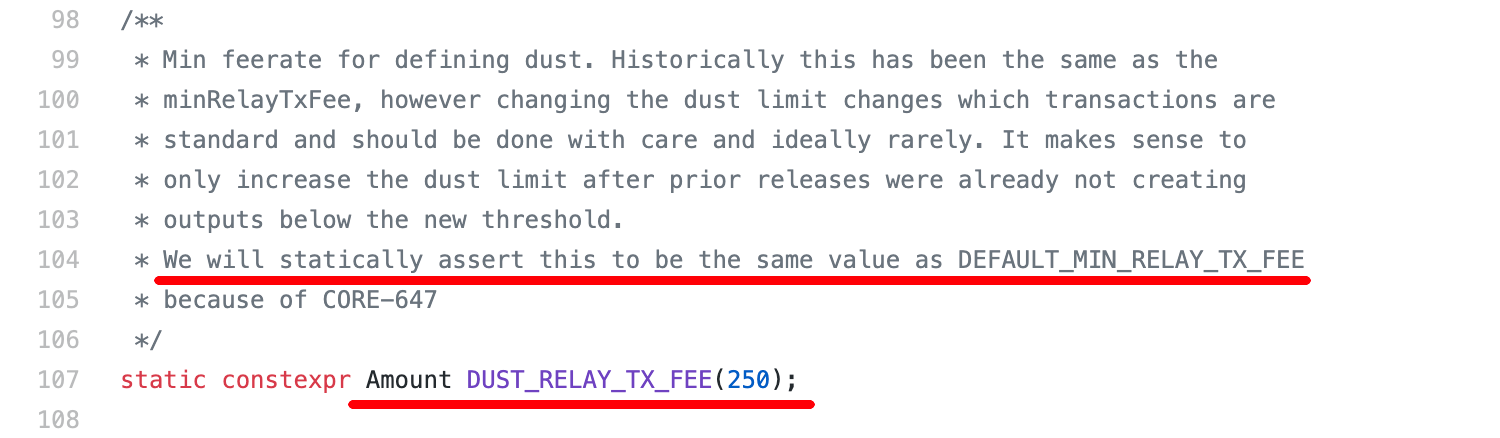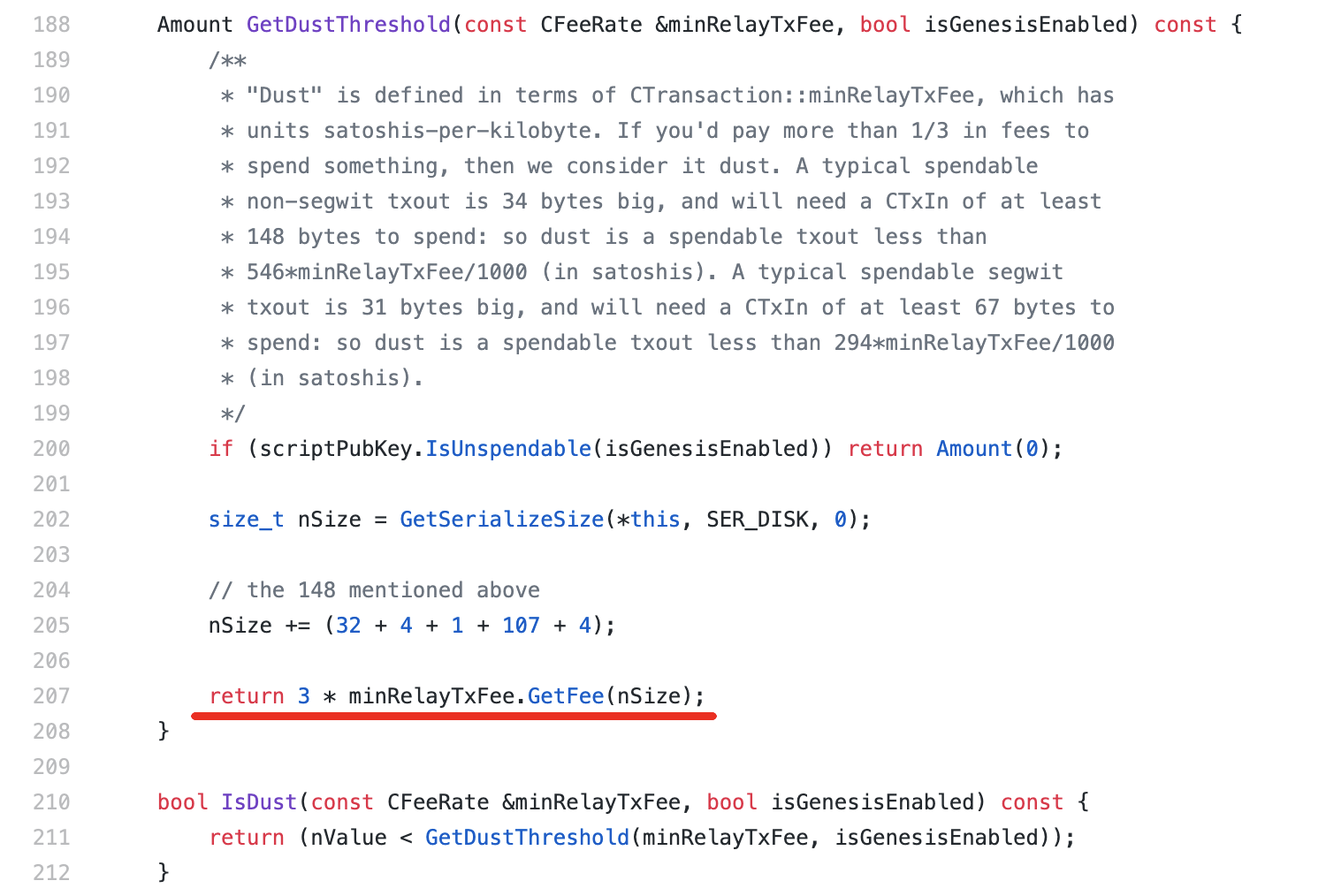所以从 1.0.5 版本开始，P2PKH 输出的 dust 将降为 135 聪。

# 验证

构造交易包含一个 135 聪的输出，在 WoC 上可以广播成功。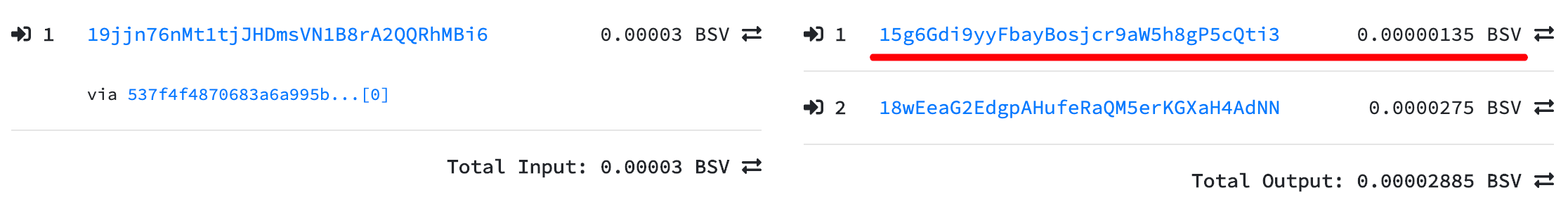构造交易包含一个 134 聪的输出，广播失败。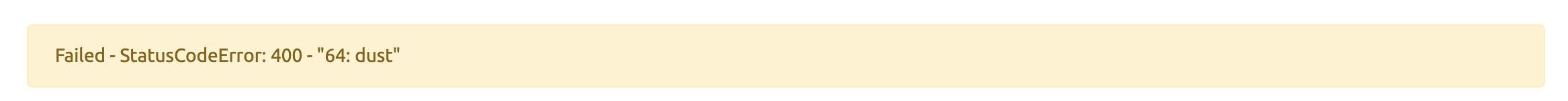原始交易为

验证结果符合预期。

]]>
<p>在第一届“打点创新营”上，我分享了有关 <a href="https://aaron67.cc/2020/01/14/dotcamp-presentation-20200104/">546</a> 的故事。</p> <p>对 Bitcoin SV 交易的 P2PKH 输出，如果其金额小于 546 聪，则该交易是粉尘交易（dust），不会被网络接受。</p> <p>从 <a href="https://bitcoinsv.io/2020/09/16/bitcoin-sv-node-software-important-upgrade-to-v1-0-5/" target="_blank" rel="noopener">1.0.5</a> 版本的节点开始，这个限制将变成 135 聪。</p>
使用比特币私钥对任意消息签名 https://aaron67.cc/2020/10/15/bitcoin-sign-arbitrary-message/ 2020-10-15T00:00:00.000Z 2023-01-25T09:43:22.860Z 在双钥系统中，签名可以作为身份认证和授权的手段。

• 对签名方，能提供正确的签名，就意味着他一定有私钥
• 对验证方，验签通过即可保证，消息内容未经篡改，且消息来源可靠

在比特币中也一样，我可以向你提供下列信息，来证明该地址属于我。

内容
地址1AfxgwYJrBgriZDLryfyKuSdBsi59jeBX9
消息你好世界
签名H9DnqMSGQmqi0zIWQqVfPXQsq59Qt11F1rUQDxv+4/iUDrSLJ6xHZ7PUSKvVThVWQAy/lLEpE3JeMpOlwUiVGlo=

使用钱包工具或第三方服务，任何人都能验证。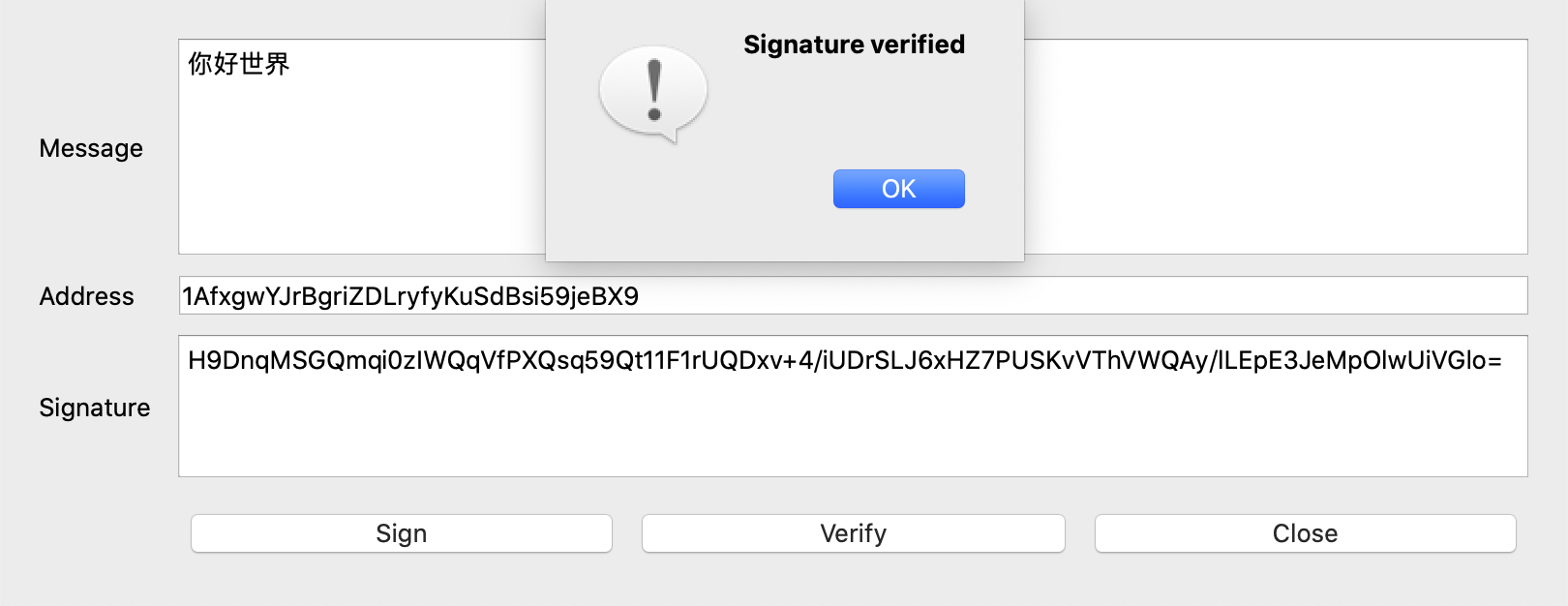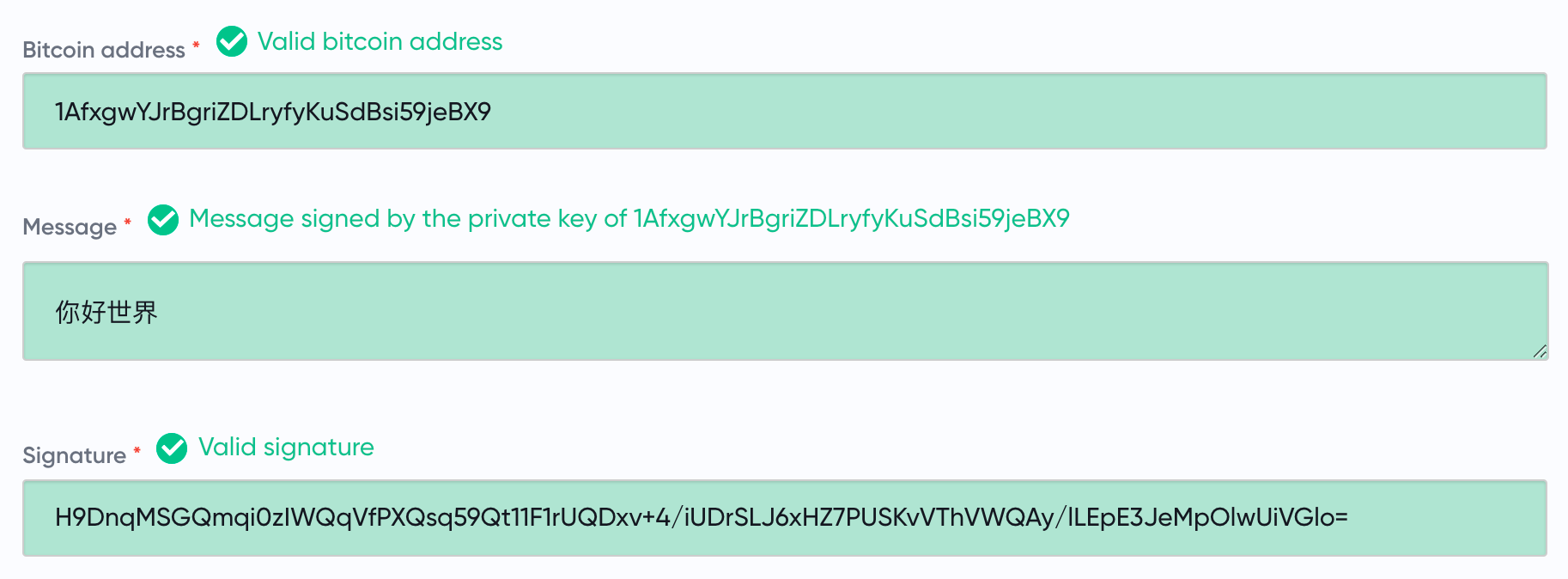验签通过即可证明，该地址对应的私钥确实在我手上。请注意，要签名的消息，可以是你我事先商议好的任何内容，这里使用“你好世界”只是演示。

本文将详述如何使用比特币私钥对任意消息签名。

# 发现“问题”

你注意到没有，文章开头的证明信息里只包含地址、消息和签名，而没有公钥。

但验签操作需要公钥。

能从地址反推吗？不能。地址是公钥哈希的可逆编码，从公钥哈希无法反推公钥，因为哈希是单向运算。所以无法从地址计算公钥。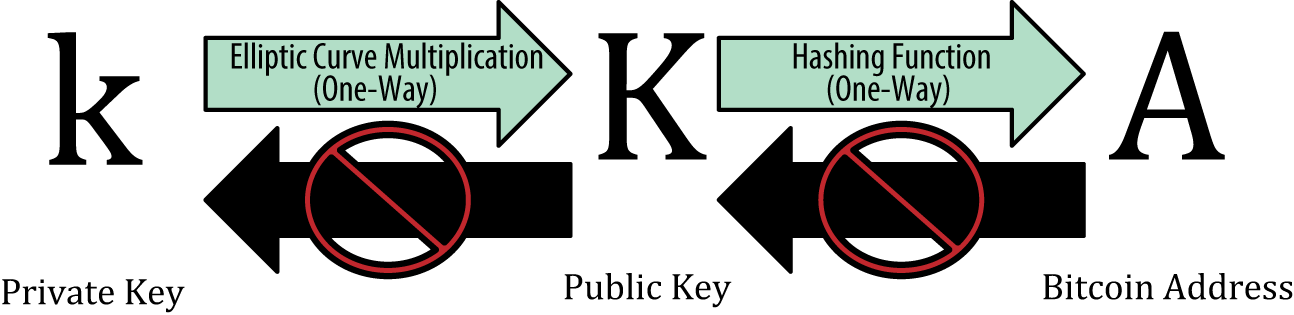那么问题来了，没有公钥如何验签？只有一种可能，从 ECDSA 签名可以恢复公钥。

# 再说 ECDSA 签名

在介绍 ECDSA 时我们强调，签名过程中使用的临时私钥 $k$，保证其绝对私密且生成时足够随机非常重要。

签名和消息是公开的，即已知 $(r, s)$ 和 $e$，如果还知道 $k$，就可以算出私钥。

$$a = r^{-1}(sk - e) \bmod{n}$$

进一步的，如果知道的是 $K$，则可以算出公钥。

$$A = aG = r^{-1}(sk - e) \cdot G = r^{-1}(s \cdot kG - eG) = r^{-1}(sK - eG)$$

所以从签名恢复公钥的关键，是确定点 $K$ 的坐标 $(x_K, y_K)$。

注意到，对 Secp256k1 的参数 $n$ 和 $p$，有 $n < p$，而 $r = x_K \bmod{n}$。

所以从 $r$ 求 $x_K$，结果不一定唯一。当 $r + n < p$ 时，$x_K = r$ 和 $x_K = r + n$ 都是解。

另外，知道了 $x_K$ 也不能唯一确定 $y_K$。椭圆曲线是上下对称的，若点 $(x, y)$ 在曲线上，则点 $(x, -y \bmod{p})$ 也在曲线上。之前的文章在介绍比特币公钥时提到过，对 Secp256k1，至少要知道点的 X 坐标和 Y 坐标的奇偶，才能唯一确定该点。

让我们总结一下。如果想通过 ECDSA 签名 $(r, s)$ 和消息 $e$ 恢复公钥，还需要知道两个额外的信息：

• $x_K$ 与 $n$ 的大小关系。若 $x_K < n$ 则 $x_K = r$，反之 $x_K = r + n$
• $y_K$ 的奇偶性

# Recoverable ECDSA 签名

这个“额外信息”只有四种状态，用 2 个二进制位即可表示。高位是 0 标记 $x_K < n$，低位是 0 标记 $y_K$ 的偶数。

二进制位内容十进制值
00$x_K < n$，$y_K$ 是偶数0
01$x_K < n$，$y_K$ 是奇数1
10$x_K > n$，$y_K$ 是偶数2
11$x_K > n$，$y_K$ 是奇数3

我们把这个标记，称为recovery_idrecid，把包含了此标记的 ECDSA 签名 $(recid, r, s)$，称为“可恢复”签名（recoverable ECDSA signature）或致密签名（compact signature）。

对应代码不难实现，只需在计算 $(r, s)$ 时同时计算recid即可。

# 计算消息摘要

用私钥对交易签名，实际上是用私钥对交易摘要签名。类似的，对消息签名时，需要先计算消息的摘要，然后对消息摘要签名。

消息摘要有特定的格式。

内容
len(H)H 的字节长度，VarInt 类型表示，定值 0x18
H字符串 Bitcoin Signed Message:\n 的 UTF-8 编码
len(M)M 的字节长度，VarInt 类型表示
M消息 $m$ 的 UTF-8 编码

调用message_digest方法，就可以得到原始消息的消息摘要。

请注意，我们在计算“消息摘要”时并没有使用哈希方法，所以严格来说这个计算的结果不能叫消息的“摘要”，对整个转换过程更准确的描述应该是“消息的格式化”或“消息的序列化”。之所以也使用“摘要”这个词，是为了将它跟之前在介绍对交易签名时提到的“交易摘要”对应起来，方便你整体理解。

# 序列化签名结果

至此，所有的准备工作均已就绪，在展示最终结果前，还需要序列化。

签名 $(recid, r, s)$ 总会被序列化成 65 字节。

字节长度内容
1前缀信息
32整数 r 按大端模式序列化后的字节流
32整数 s 按大端模式序列化后的字节流

别忘了，比特币公钥有两种表示法，一种以 0x04 开头有 65 字节，另一种以 0x02 或 0x03 开头有 33 字节，即同一个公钥会对应两个 P2PKH 地址。为了能在验签时同时校验恢复出的公钥的哈希，是否与输入的地址相匹配，还需要在前缀信息里标记公钥的表示方式。

前缀的值内容
27 + recid + 4公钥用 33 字节表示
27 + recid + 0公钥用 65 字节表示

最后，用 Base64 编码处理序列化后的签名数据，以方便人们复制和转录。

我们写一个简单的例子来测试。

输出为

使用钱包工具或第三方服务验证这个输出，可以验签成功。# 验签

在验证对消息的签名时，输入变成了比特币地址、原始消息和序列化后的致密签名，所以对应的验签过程也要微调。

1. 从序列化后的签名中，解析出recid和 $(r, s)$，同时明确公钥的表示方式
2. 恢复公钥
3. 用公钥和消息验证签名 $(r, s)$
4. 验证恢复出的公钥的哈希，是否与输入的比特币地址相匹配

利用recid恢复公钥时，需要从点的 X 坐标求对应的 Y 坐标。对 Secp256k1 的曲线方程

$$y^2 \equiv x^3 + 7 \pmod{p}$$

知道了 $x$ 就等于知道了 $y^2 \bmod{p}$，为了求 $y$，需要“开方”，这很麻烦，因为有模运算。我们可以用费马小定理来改进。

对整数 $a$ 和质数 $p$，有

$$a^p \equiv a \pmod{p}$$

曲线 Secp256k1 的 $p$ 是一个质数，所以有

$$y^{p} \equiv y \pmod{p}$$

利用同余关系的性质，将上式两边同时放大 $y$ 倍，有

$$y^{p+1} \equiv y^2 \pmod{p}$$

$$(y^2)^{\frac{p+1}{2}} \equiv y^2 \pmod{p}$$

$$y = (y^2)^{\frac{p+1}{4}} \bmod{p}\ \ \ \ 或\ \ \ \ -(y^2)^{\frac{p+1}{4}} \bmod{p}$$

注意到，$\frac{p+1}{4}$ 是一个整数。也就是说，通过计算 $y^2$ 的整数次幂，就可以求解 $y$。

有了这些知识，不难写出对应的验签代码，请注意求解point_k时对费马小定理的应用。

我们用其他工具生成的签名作为输入，来检验验签代码的正确性。将私钥导入 ElectrumSV 钱包对消息签名，可以得到结果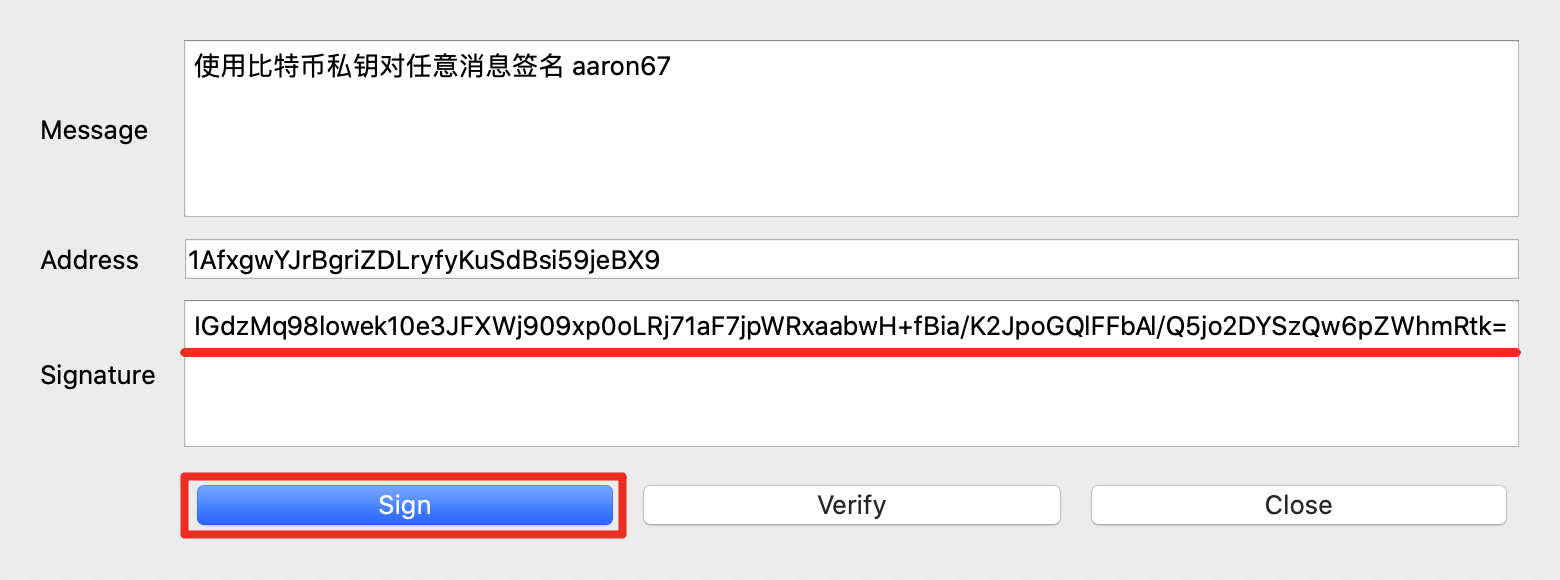调用验签方法测试。

运行结果为

输出符合预期。

# 完整代码

sign_message.py

# 参考

]]>
<p>在双钥系统中，签名可以作为身份认证和授权的手段。</p> <ul> <li>对签名方，能提供正确的签名，就意味着他一定有私钥</li> <li>对验证方，验签通过即可保证，消息内容未经篡改，且消息来源可靠</li> </ul> <p>在比特币中也一样，我可以向你提供下列信息，来证明该地址属于我。</p> <table> <thead> <tr> <th>项</th> <th>内容</th> </tr> </thead> <tbody> <tr> <td>地址</td> <td>1AfxgwYJrBgriZDLryfyKuSdBsi59jeBX9</td> </tr> <tr> <td>消息</td> <td>你好世界</td> </tr> <tr> <td>签名</td> <td>H9DnqMSGQmqi0zIWQqVfPXQsq59Qt11F1rUQDxv+4/iUDrSLJ6xHZ7PUSKvVThVWQAy/lLEpE3JeMpOlwUiVGlo=</td> </tr> </tbody> </table>
比特币交易中的签名 https://aaron67.cc/2020/10/10/bitcoin-sign-transaction/ 2020-10-10T00:00:00.000Z 2023-01-25T09:43:22.860Z 比特币以 UTXO 的形式“存储”在全网账本中，被放置在其上的加密难题（锁定脚本）锁定，只有（要）提供正确的解锁脚本解决或满足这个加密难题或条件，才（就）可以用于支付。

结合丰富的操作码，锁定脚本和解锁脚本的形式拥有广泛的可能性。当锁定脚本为OP_ADD 7 OP_EQUAL时，5 24 3都是正确解锁脚本。当蔡明使用比特币收款时，她需要提供一个收款模板（锁定脚本），以确保这些比特币只有自己才能花费。很明显，上述这类锁定脚本与现实世界人的身份毫无关联，虽然蔡明可以想方设法将锁定脚本搞的足够复杂，但这种方式并不通用，更没有安全保障，无法彻底杜绝其他人也能提供正确的解锁脚本。

非对称加密中的公钥可以作为身份标识，签名可以作为身份认证和授权的手段。为了做到这一点，蔡明需要在锁定脚本里关联自己的公钥，并限制只有提供了正确的数字签名才能花费这个 UTXO。数学原理可以保证，只要蔡明的私钥没有丢失或泄露就没有其他人能提供正确的签名。

上述这类交易被统称为 P2PKH 交易（P2PK 的演进版），他们的锁定脚本和解锁脚本格式固定，能方便各类钱包集成。比特币网络中的绝大多数交易都是（郭达付款给蔡明）这样的形式，本文将以 P2PKH 交易为例，详细介绍交易签名的细节。

# 序列化 ECDSA 签名

之前的文章介绍了如何创建 ECDSA 签名。在把 $(r, s)$ 放入解锁脚本前，需要先将其序列化成 DER 格式，结构如下。

字节长度内容
1格式头 0x30
1紧跟其后的所有数据的总长度
1整数标志 0x02
1R 的长度
变长整数 r 按大端模式序列化后的字节流 R。当流的起始字节不小于 0x80 时，还需要在流的开头添加 0x00
1整数标志 0x02
1S 的长度
变长整数 s 按大端模式序列化后的字节流 S。当流的起始字节不小于 0x80 时，还需要在流的开头添加 0x00

根据定义不难写出代码。请注意序列化时对 S 的处理，因为 ECDSA 签名的“对称性”，为了避免“混乱”，会强制 DER 中的 S 不大于

curve.n // 2

我们写一个简单的例子来测试。

运行结果为

# 准备工作

在继续之前，我们需要先实现一些基础方法，限于篇幅，请直接参考完整代码。

• crypto.py，包含常用的哈希算法和 Base58Check 编解码方法等
• meta.py，包含int_to_varintaddress_to_public_key_hashbuild_locking_script等常用方法，这些内容在之前的“学习笔记”系列文章中都有过介绍

顺便封装一下交易的输入和输出。

# 交易摘要

验证 ECDSA 签名是否有效，需要三个参数：

• 消息
• ECC 公钥
• ECDSA 签名

如果你还记得 P2PKH 的定义，你会发现，不论是解锁脚本还是锁定脚本，都没有明确被签名的消息是什么。

请注意，解锁脚本中的签名，其实由两部分构成。

字节长度内容
1紧跟其后的所有数据的总长度
变长序列化后的 ECDSA 签名
1SIGHASH

之前的文章提到过，交易中被签名的消息，是交易本身。更准确的说，是通过 SIGHASH 标记区分的、交易中特定的数据子集

交易本身在签名和验签时是已知的，也就是说，虽然脚本中没有直接存储消息的内容，但存储了能间接推算出消息内容的 SIGHASH。这个“推算出的消息内容”，叫交易的摘要。

我们需要实现一个方法，根据交易和 SIGHASH 来计算交易的摘要。

SIGHASH 有 6 不同的类型：

• SIGHASH_ALL
• SIGHASH_NONE
• SIGHASH_SINGLE
• SIGHASH_ALL | ANYONECANPAY
• SIGHASH_NONE | ANYONECANPAY
• SIGHASH_SINGLE | ANYONECANPAY

全网几乎所有的交易都使用 SIGHASH_ALL，这是最简单的一种类型，我们将以此为例。其他类型的 SIGHASH 本文暂不涉及，你可以通过文章 SIGHASH flagsBIP-143 探索。

请注意，SIGHASH_ALL 会对所有的交易输入签名，也就是说，对应交易摘要的个数，与交易输入的个数相同。

# 实验

让我们在之前的例子上继续。私钥

锁定了 3 个 UTXO。

## 验证已签名的交易

事先使用其他钱包 App，消耗inputs，向地址1JDZRGf5fPjGTpqLNwjHFFZnagcZbwDsxw支付 800 聪，对应的交易是

基于此场景，让我们开始第一个实验：验证这个已签名交易中的 ECDSA 签名。

公钥已知，为了验签，还需要利用这个交易

• 计算出交易摘要，得到要签名的消息
• 反序列化 DER 签名，得到 $(r, s)$

开始吧。

1. 构造输入和输出
1. 根据交易的输入和输出计算交易摘要（使用 SIGHASH_ALL）
1. 反序列化签名，得到 $(r, s)$

通过区块链浏览器，查询序列化后的交易数据。图中标注的部分，是序列化后的 ECDSA 签名，通过之前实现的方法反序列化。

1. 验签

运行结果为

验签成功。## 创建交易并签名

第二个小实验，我们用自己实现的代码，创建交易并对其签名。如果交易广播后比特币网络能正常接受，那么说明我们的代码是正确的。

这个交易会将inputsinputs作为输入，向地址18CgRLx9hFZqDZv75J5kED7ANnDriwvpi1支付 1700 聪。

开始吧。

1. 构造输入和输出，并计算交易摘要
1. 对每个交易摘要签名，并且构造对应的解锁脚本
1. 根据输入（已签名）和输出构造完整的交易

序列化后的交易格式在“学习笔记”系列文章中有过详细介绍，这里也列出来方便你对应代码。

字节长度内容
4交易结构的版本
1~9 VarInt交易包含几个输入，非零正整数
变长输入数组
1~9 VarInt交易包含几个输出，非零正整数
变长输出数组
4nLockTime

将序列化后的交易数据打印出来，广播时会用到。同时计算交易的哈希。

1. 验证

代码的运行结果为

其中，第一行和第三行是两个交易输入的解锁脚本，倒数第二行是序列化后的交易，最后一行是这个交易的哈希。

我在 WhatsOnChain 上，正常广播了这个交易。请注意下图标注的部分，浏览器解析后的解锁脚本，跟我们的计算结果是相同的。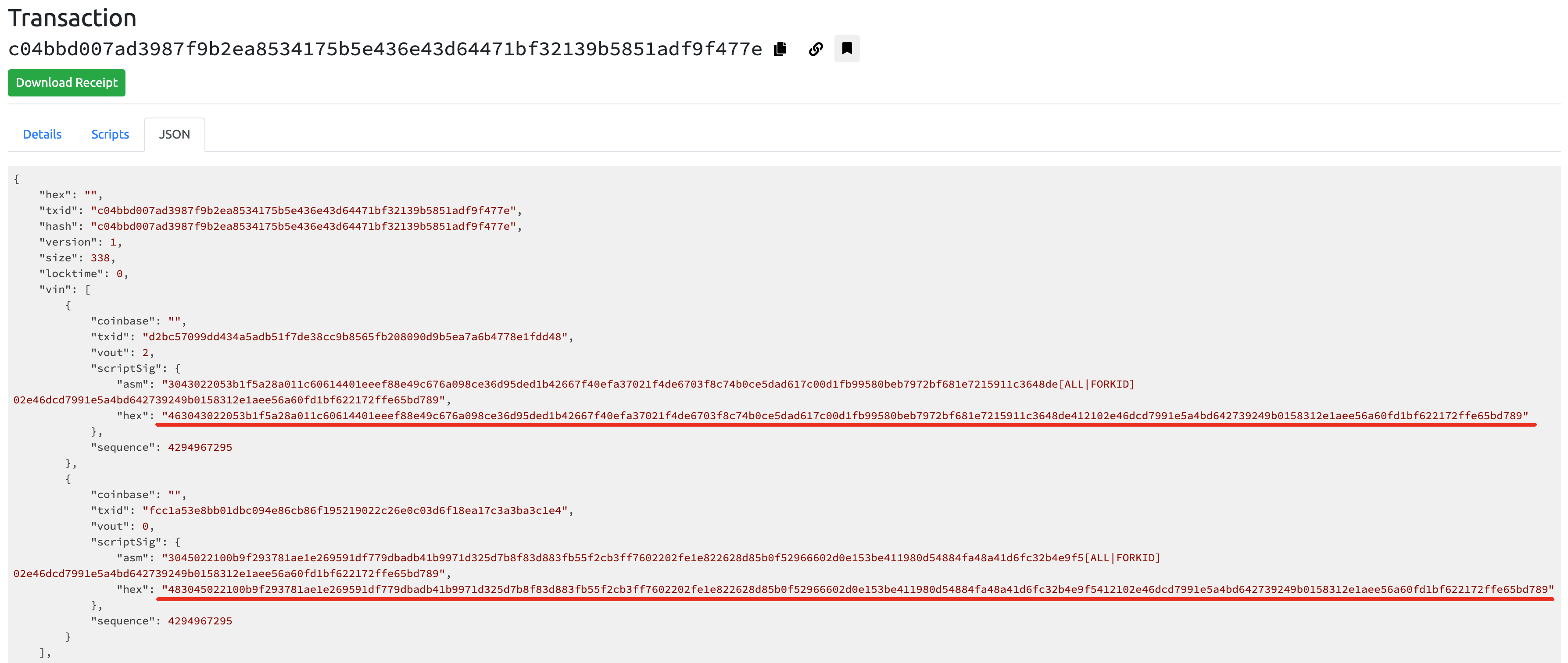至此，实验成功。# 完整代码

sign_transaction.py

# 参考

]]>
<p>比特币以 <a href="https://aaron67.cc/2018/12/25/bitcoin-transaction-primer/#UTXO">UTXO</a> 的形式“存储”在全网账本中，被放置在其上的加密难题（锁定脚本）锁定，只有（要）提供正确的解锁脚本解决或满足这个加密难题或条件，才（就）可以用于支付。</p> <p>结合丰富的<a href="https://en.bitcoin.it/wiki/Script#Opcodes" target="_blank" rel="noopener">操作码</a>，锁定脚本和解锁脚本的形式拥有广泛的可能性。当锁定脚本为<code>OP_ADD 7 OP_EQUAL</code>时，<code>5 2</code>和<code>4 3</code>都是正确解锁脚本。当蔡明使用比特币收款时，她需要提供一个收款模板（锁定脚本），以确保这些比特币只有自己才能花费。很明显，上述这类锁定脚本与现实世界人的身份毫无关联，虽然蔡明可以想方设法将锁定脚本搞的足够复杂，但这种方式并不通用，更没有安全保障，无法彻底杜绝其他人也能提供正确的解锁脚本。</p> <p>非对称加密中的公钥可以作为身份标识，签名可以作为身份认证和授权的手段。为了做到这一点，蔡明需要在锁定脚本里关联自己的公钥，并限制只有提供了正确的数字签名才能花费这个 UTXO。数学原理可以保证，只要蔡明的私钥没有丢失或泄露就没有其他人能提供正确的签名。</p> <p>上述这类交易被统称为 <a href="https://aaron67.cc/2018/12/28/bitcoin-transaction-p2pk-p2pkh/">P2PKH</a> 交易（P2PK 的演进版），他们的锁定脚本和解锁脚本格式固定，能方便各类钱包集成。比特币网络中的绝大多数交易都是（郭达付款给蔡明）这样的形式，本文将以 P2PKH 交易为例，详细介绍交易签名的细节。</p>